# Lakhmir Singh Solutions Class 9 Physics Chapter 1 Motion

Motion is defined as the movement of an object from one place to another. When an object moves along a straight line, the motion is known as rectilinear motion. Distance, displacement, uniform motion, non-uniform motion, speed, acceleration and velocity are the terms that are used for explaining the motion. Motion can also be represented with the help of graphs. There are different types of graphs that are used and they are:

• Distance-time graph for uniform and non-uniform speed of an object and when the object is at rest.
• Velocity-time graph for uniform and non-uniform acceleration of an object.

The motion of an object can be of different types, depending on the type of path the object is moving through and they are:

• Linear motion: When the object moves along a straight path.
• Circular motion: When the object moves in a circular path.
• Oscillatory motion: When the object is moving in a to and fro path with respect to its origin.

Scalar and vector are the two quantities that are used in motion. A quantity is said to be a scalar quantity when it has a magnitude alone. Distance and speed are examples of scalar quantities. When a quantity has magnitude and direction, the quantity is said to be a vector quantity. Velocity and displacement are examples of vector quantities.

## Download PDF Of Lakhmir Singh Physics Class 9 Solutions For Chapter 1 Motion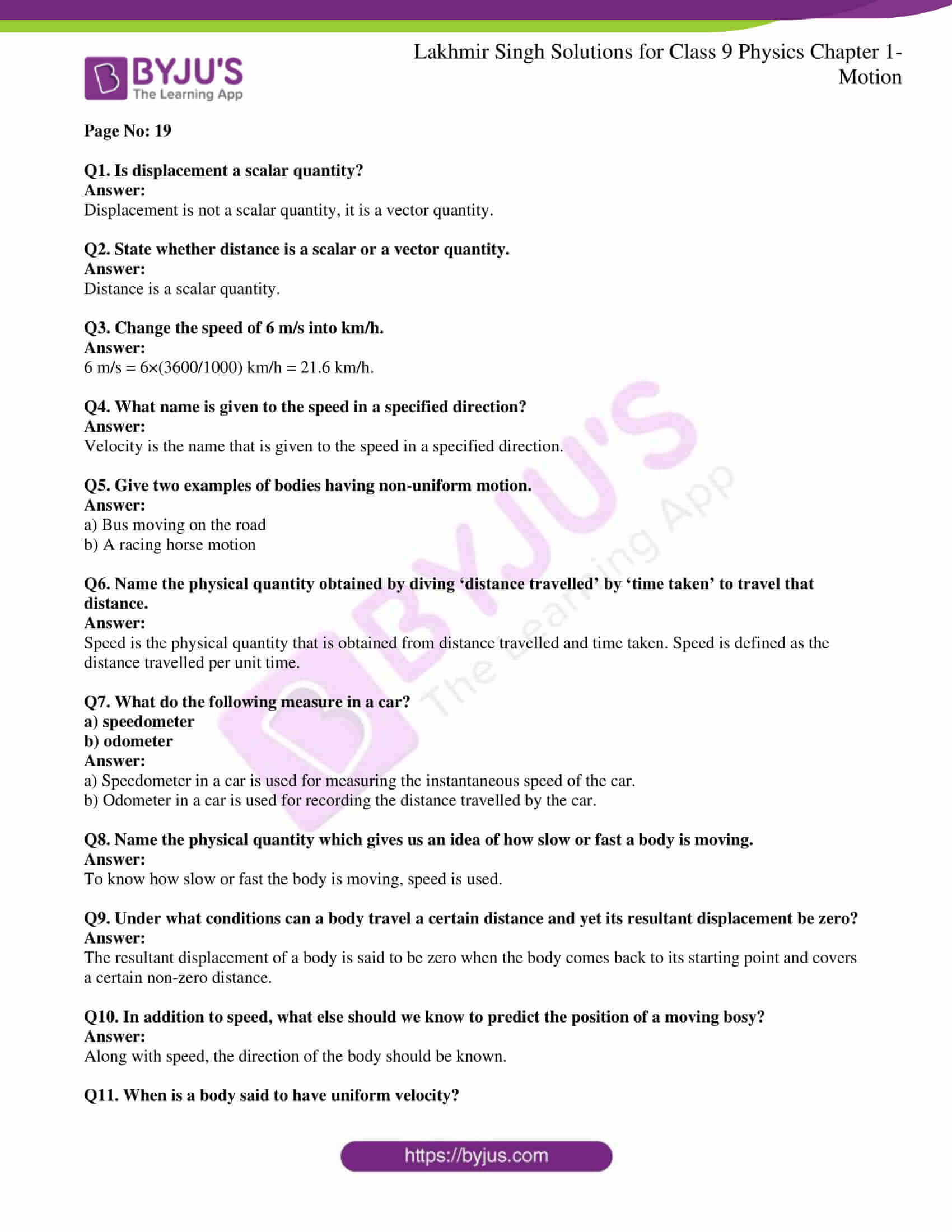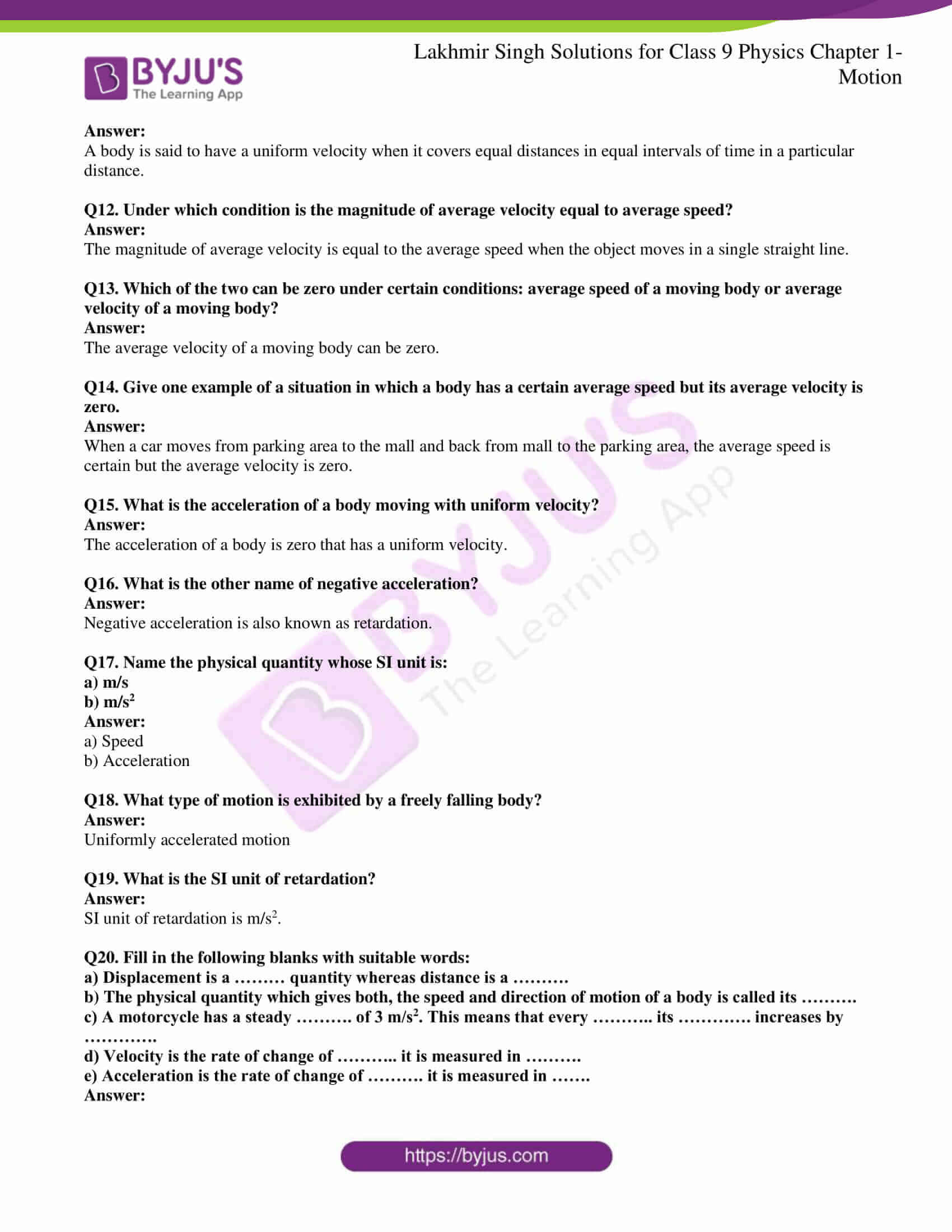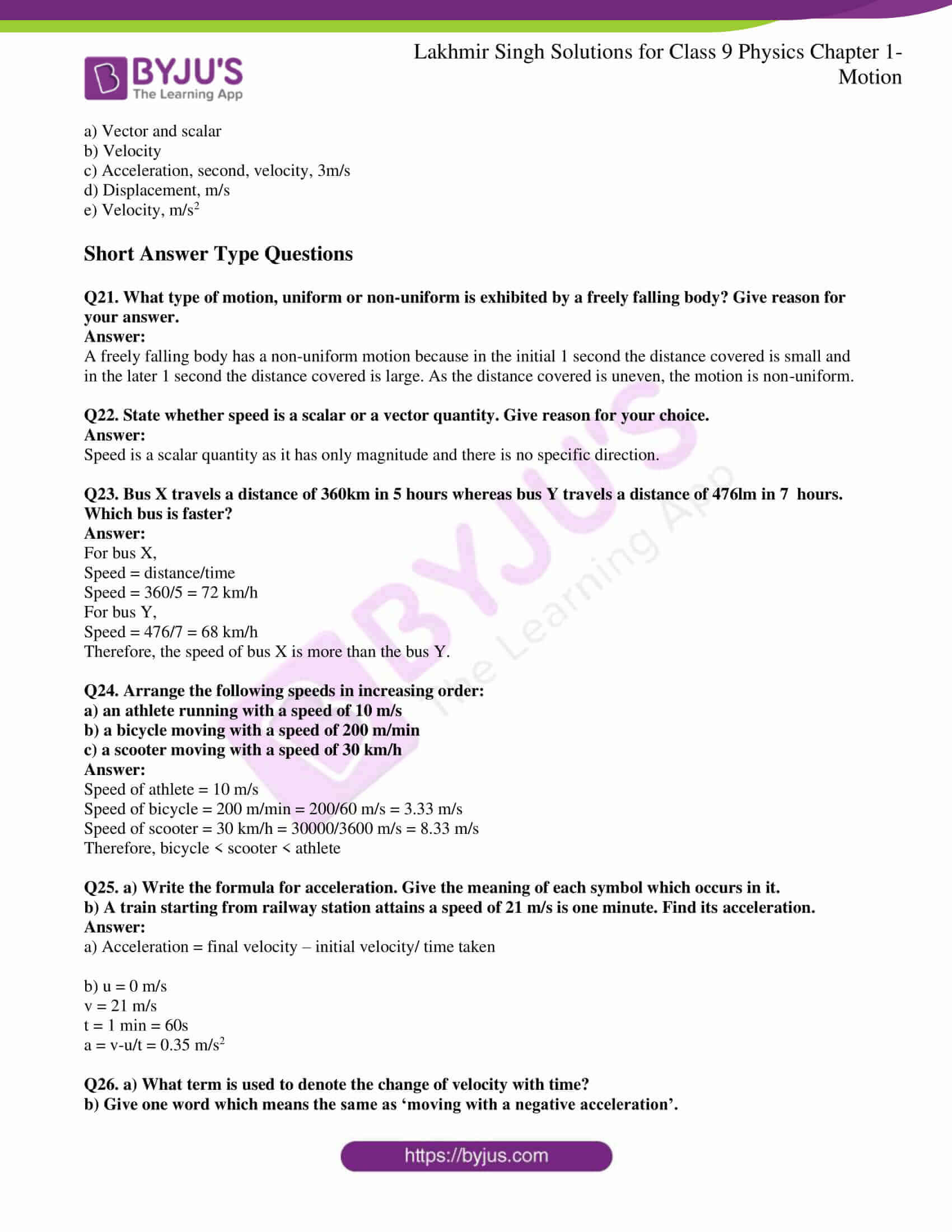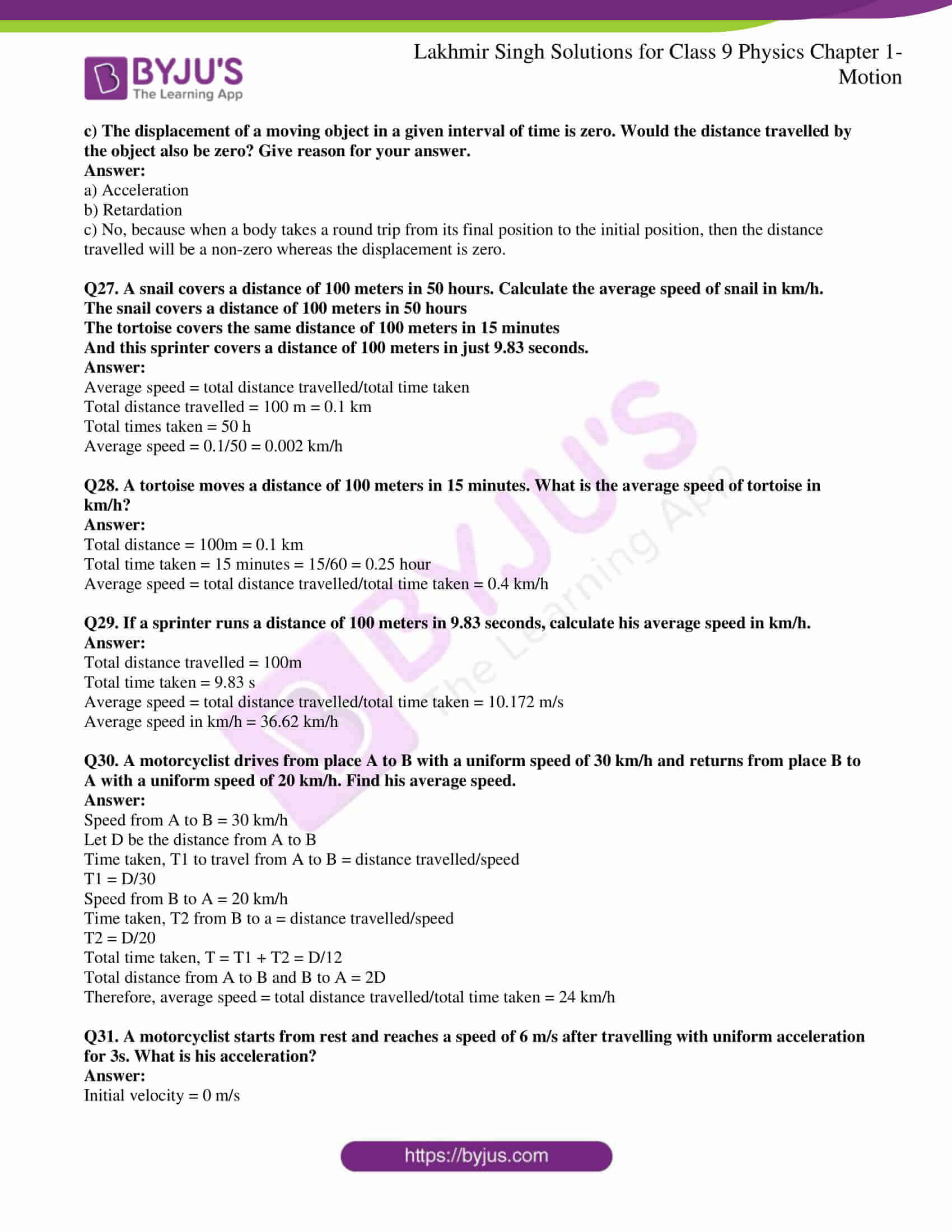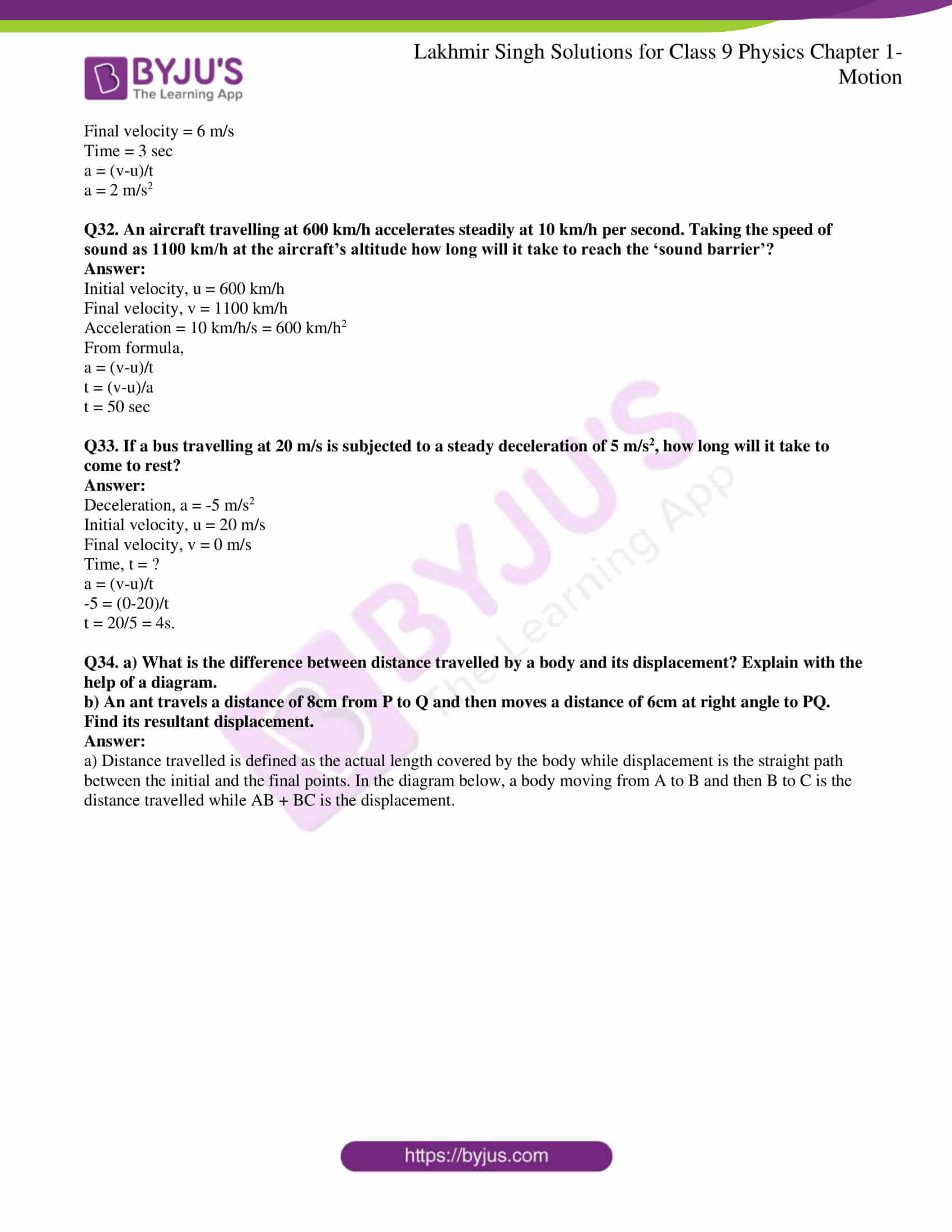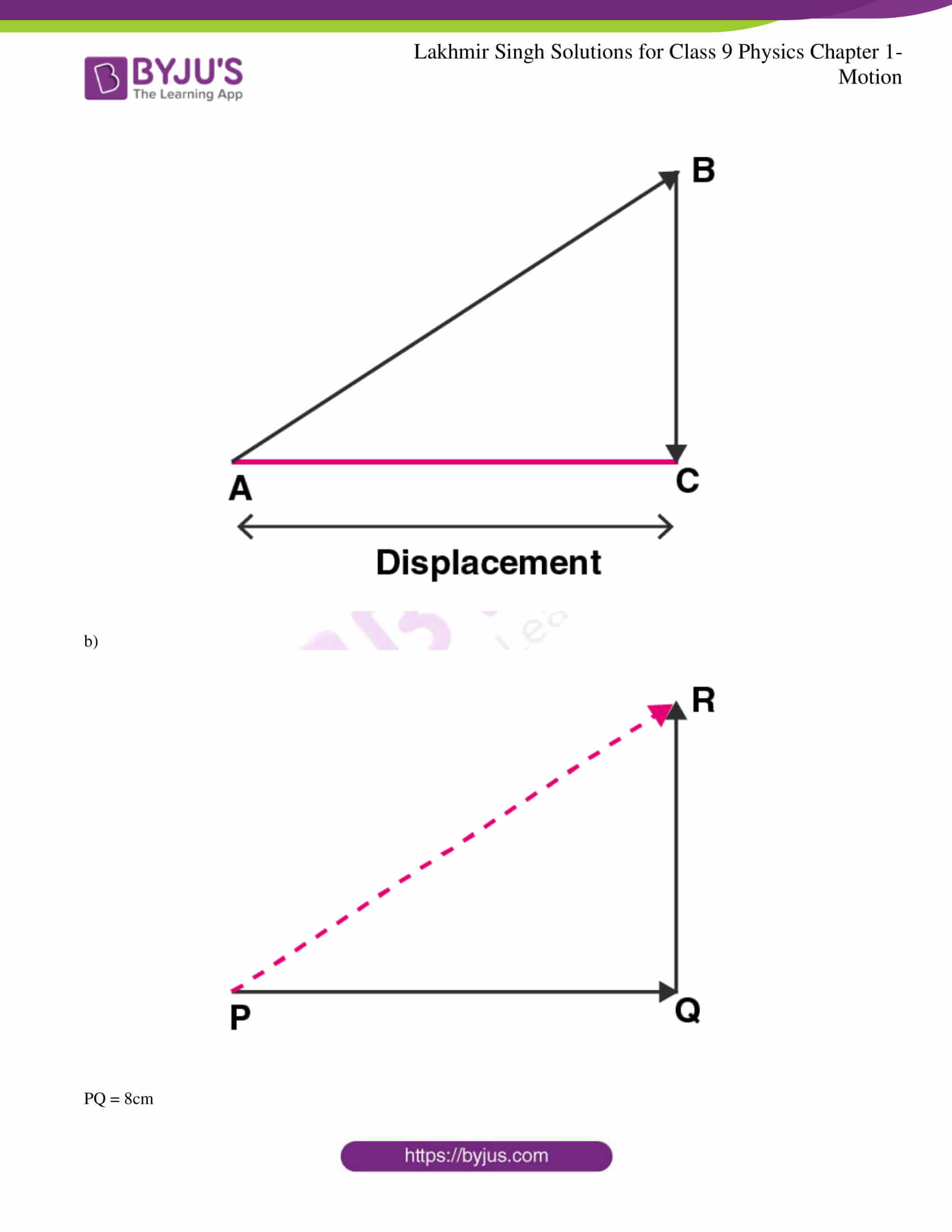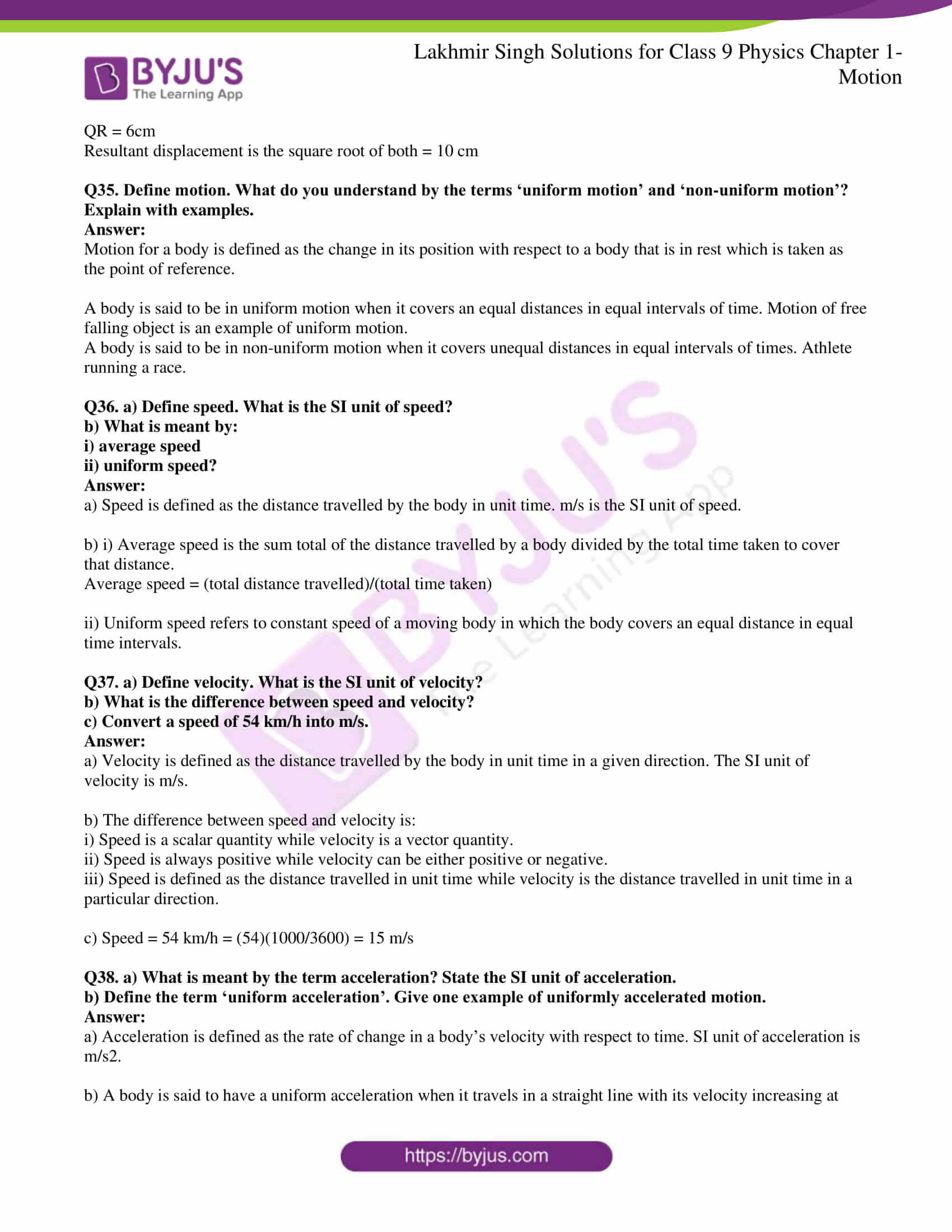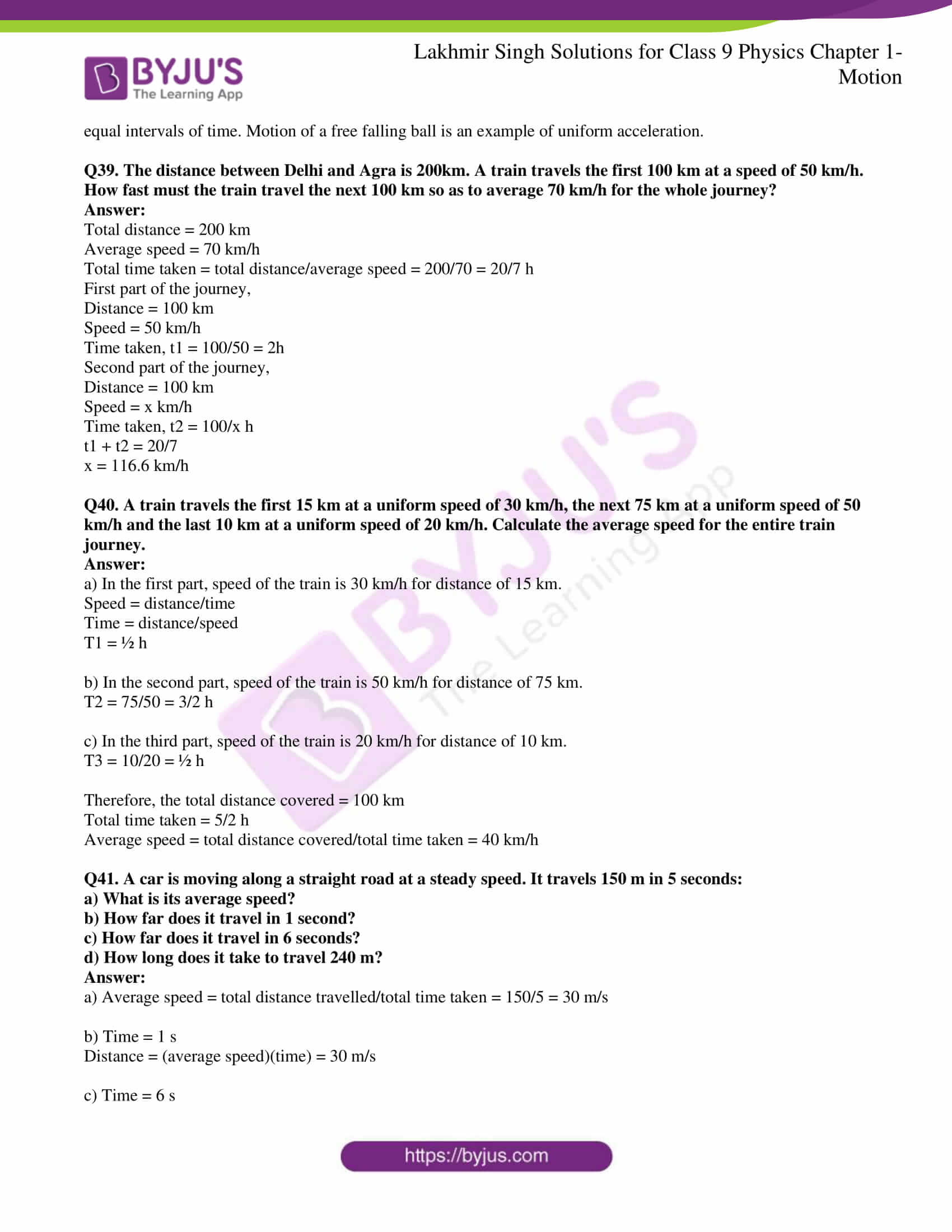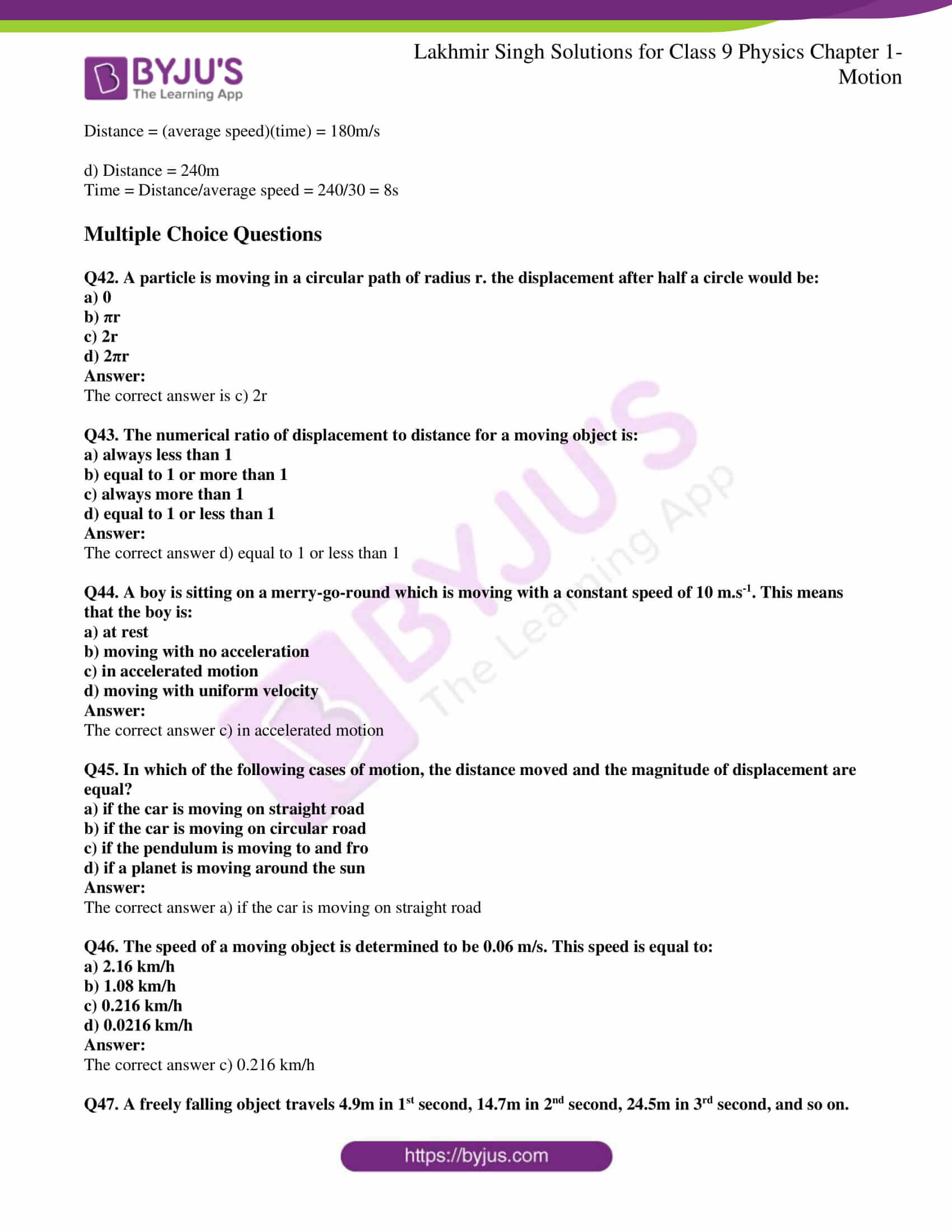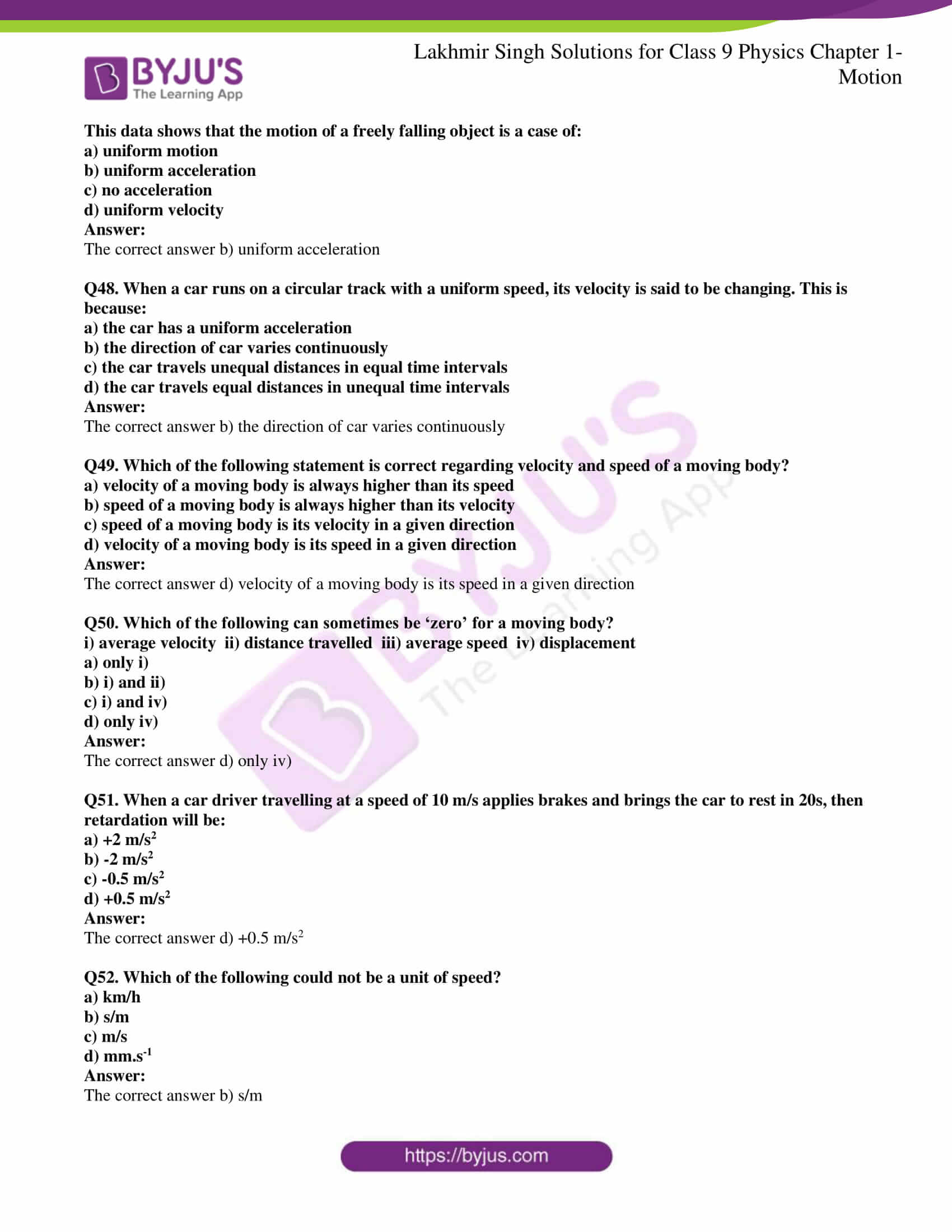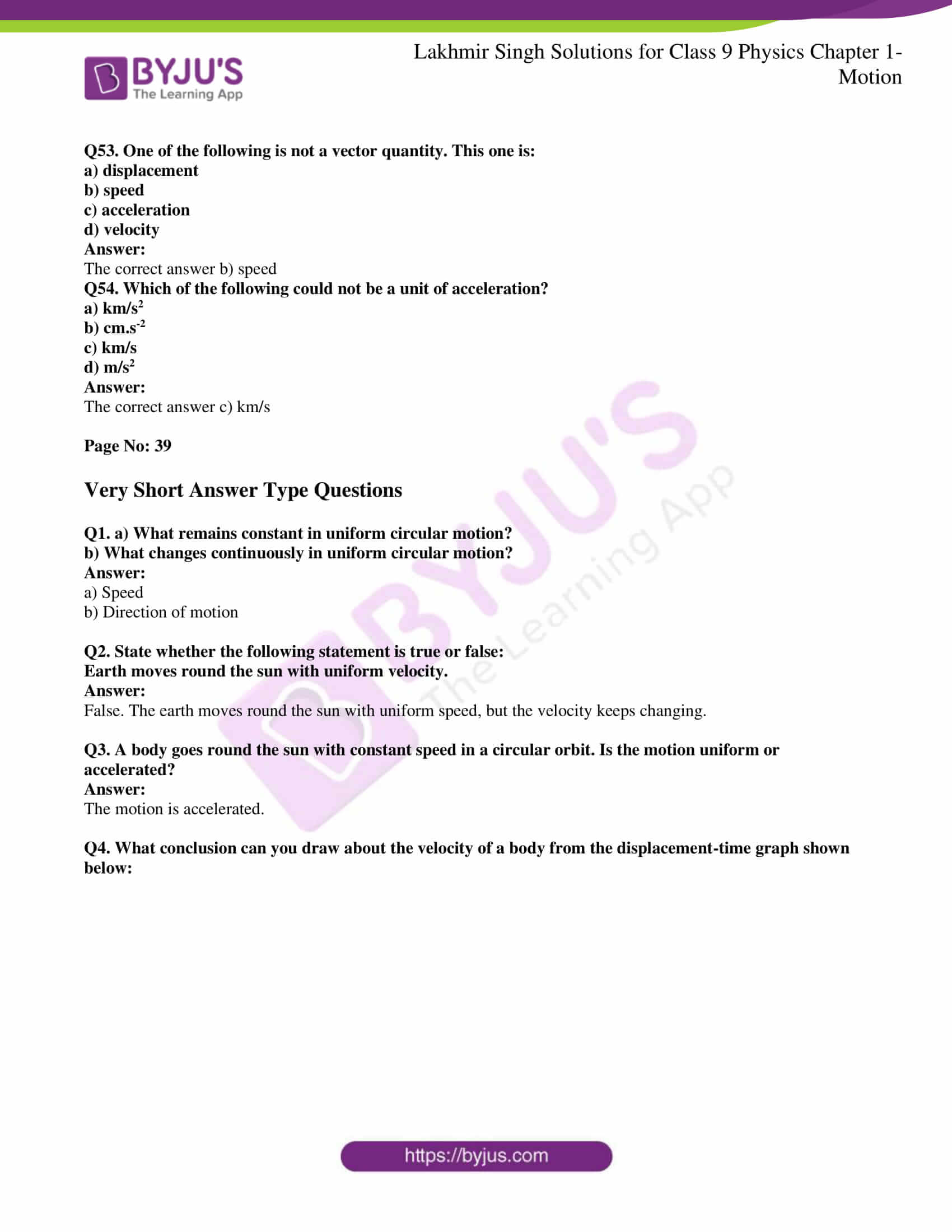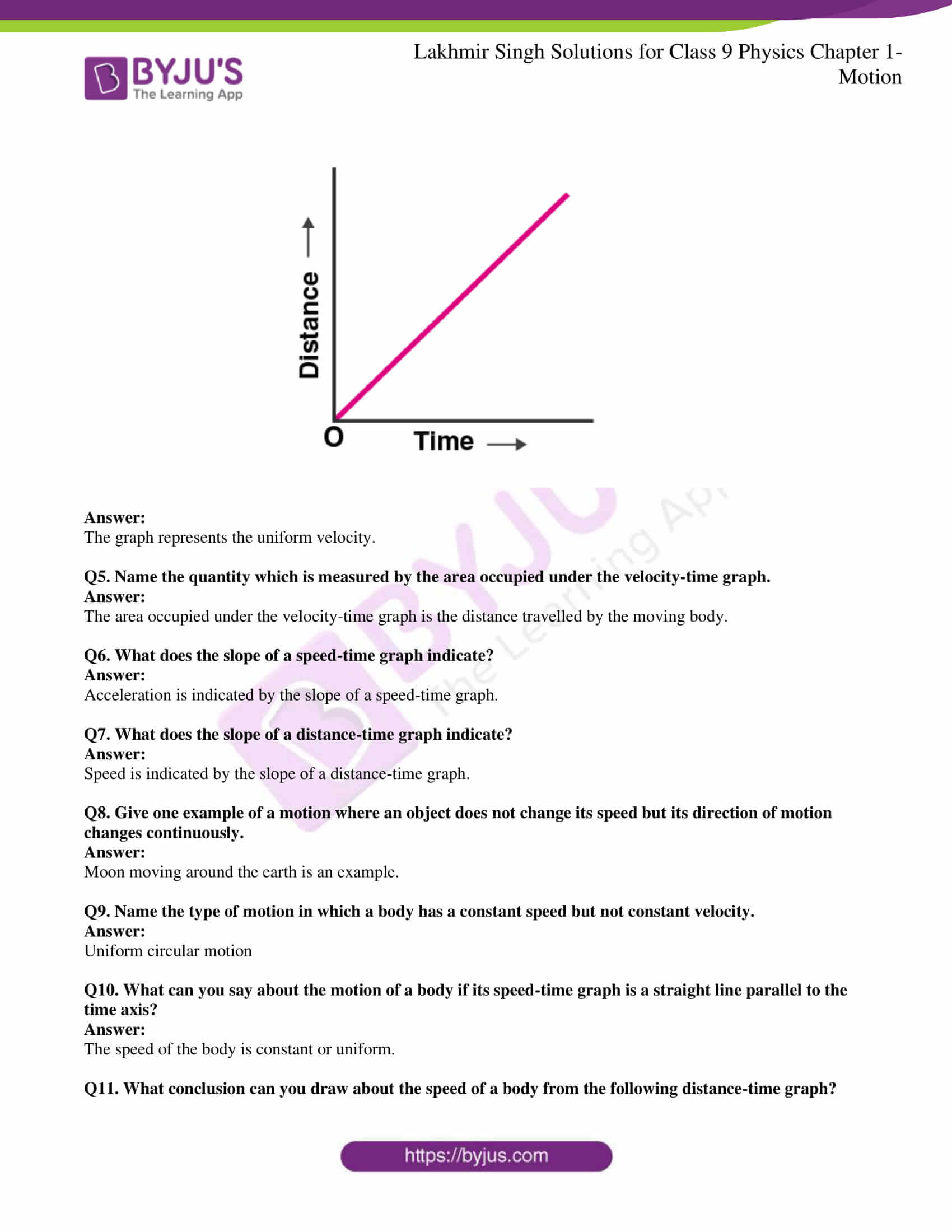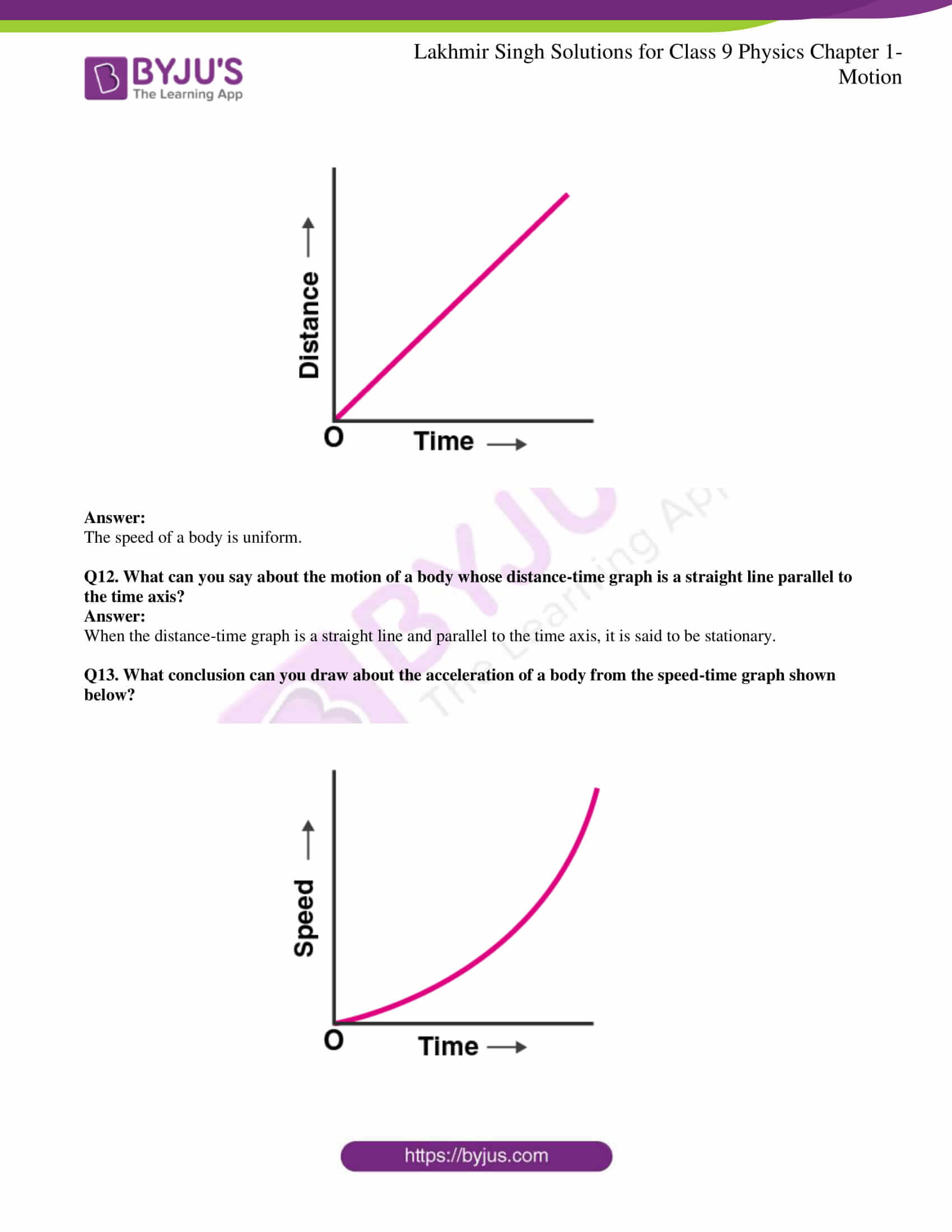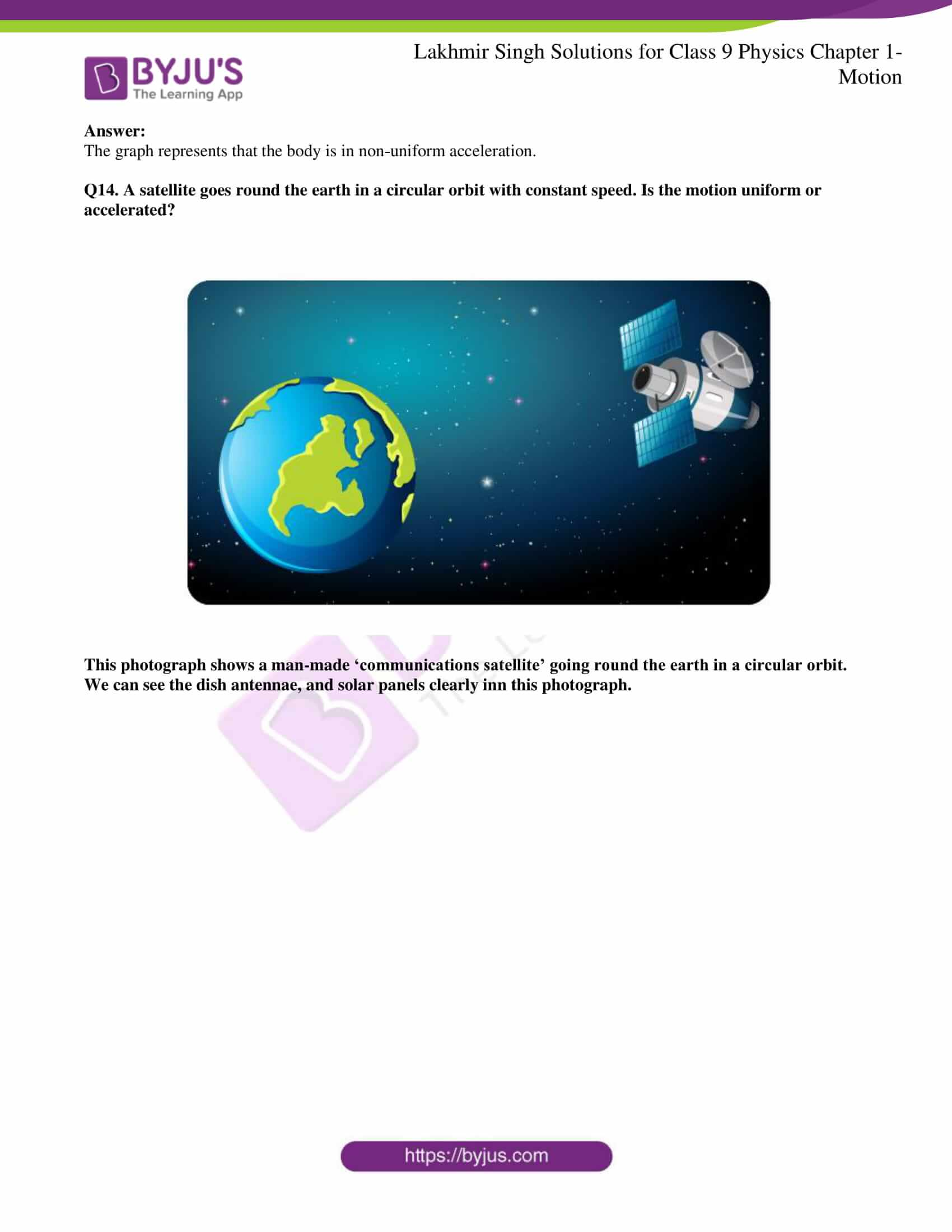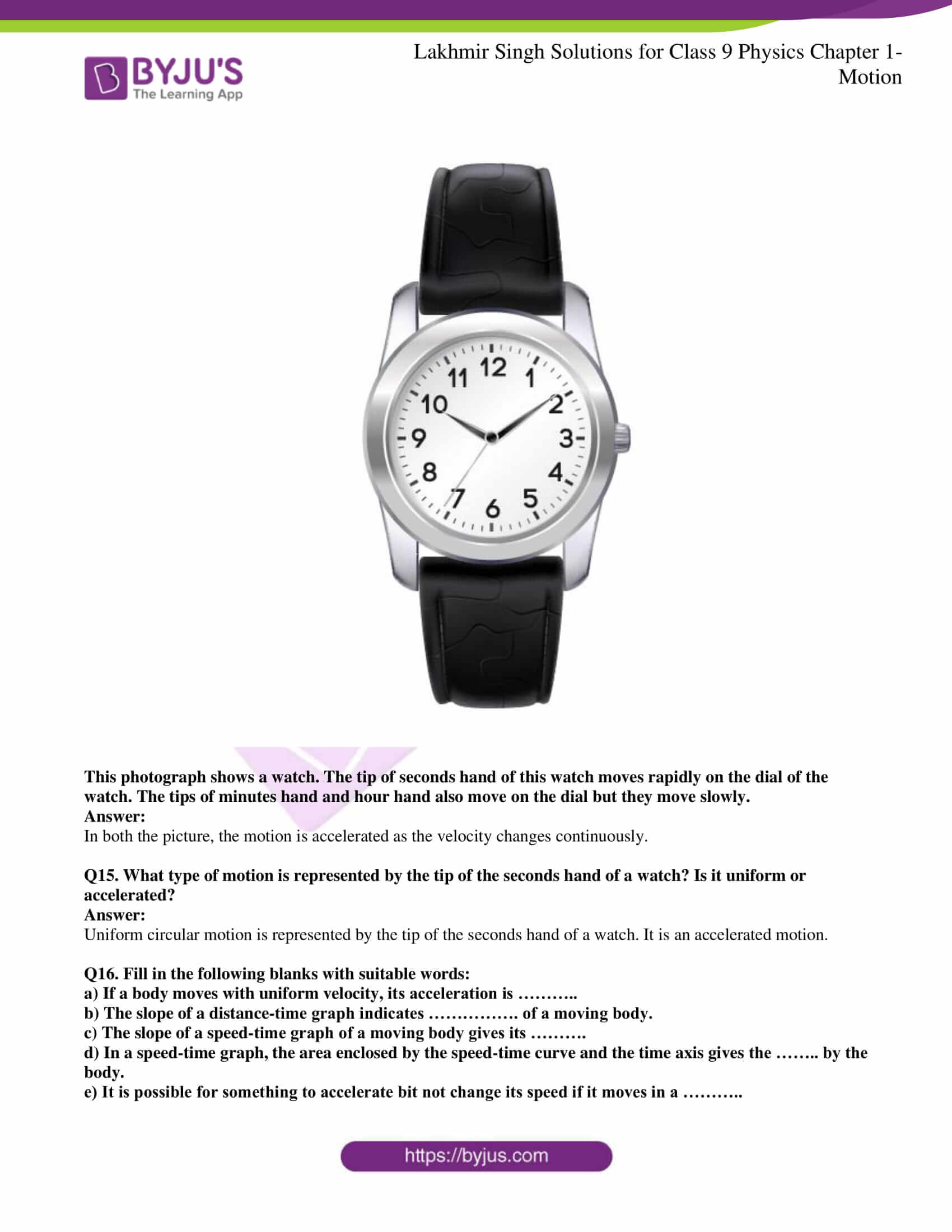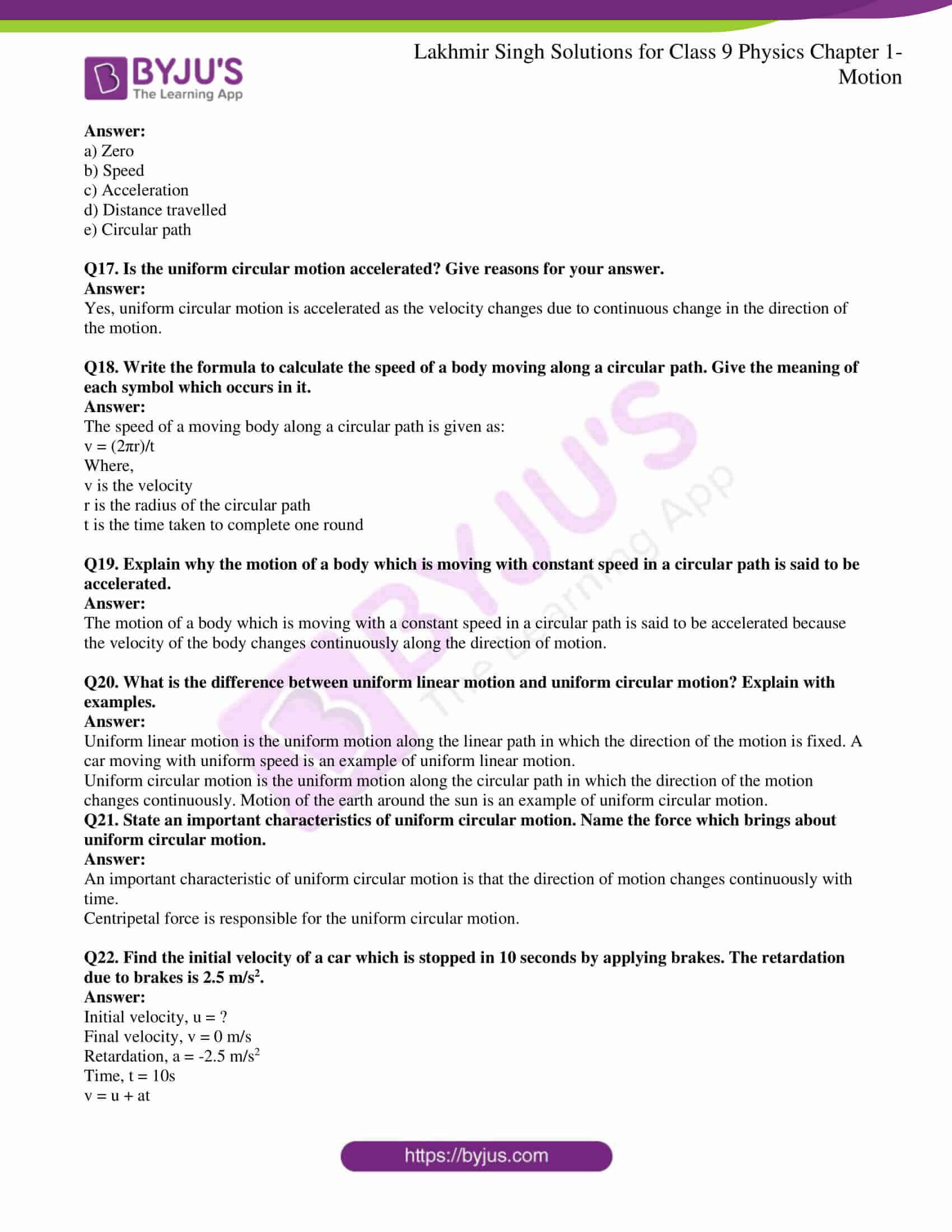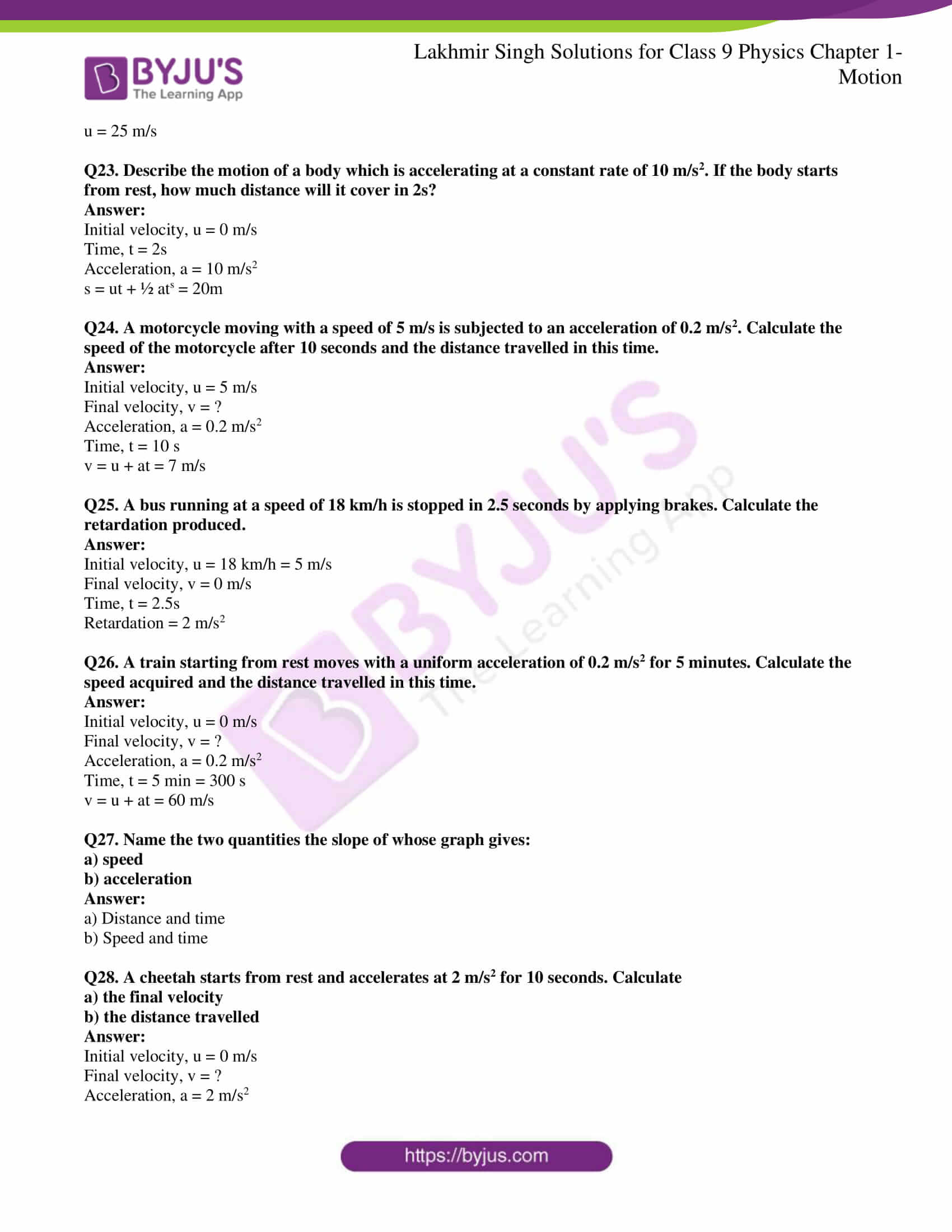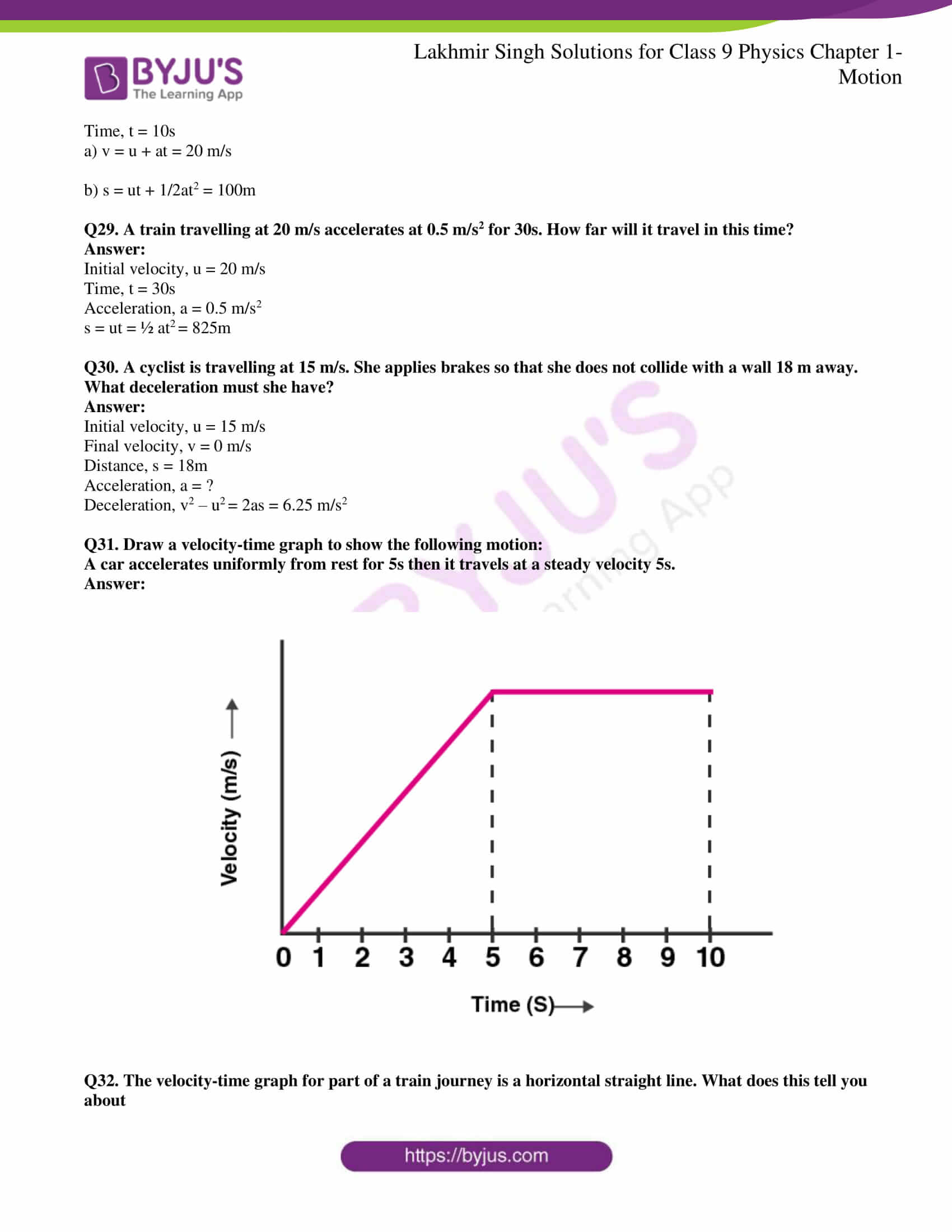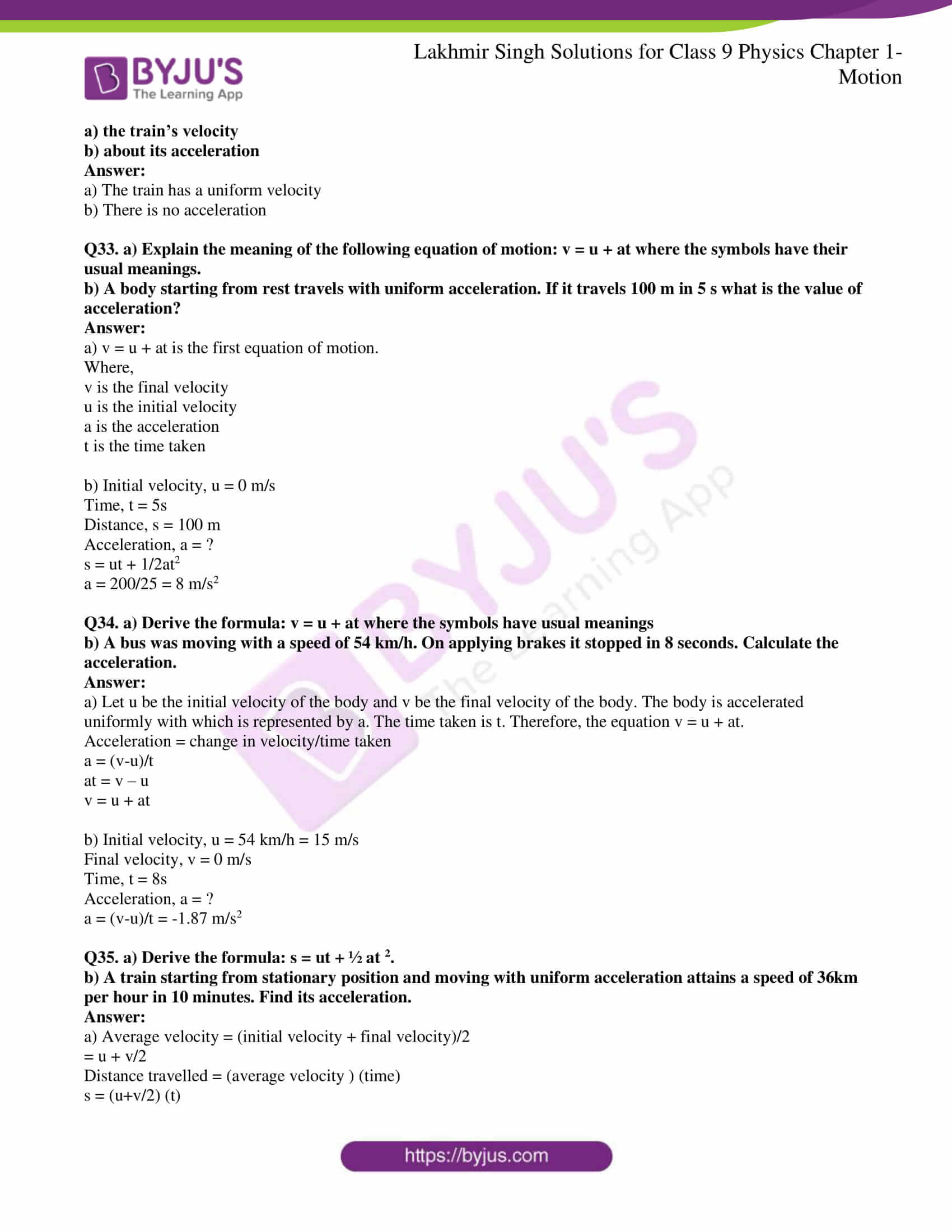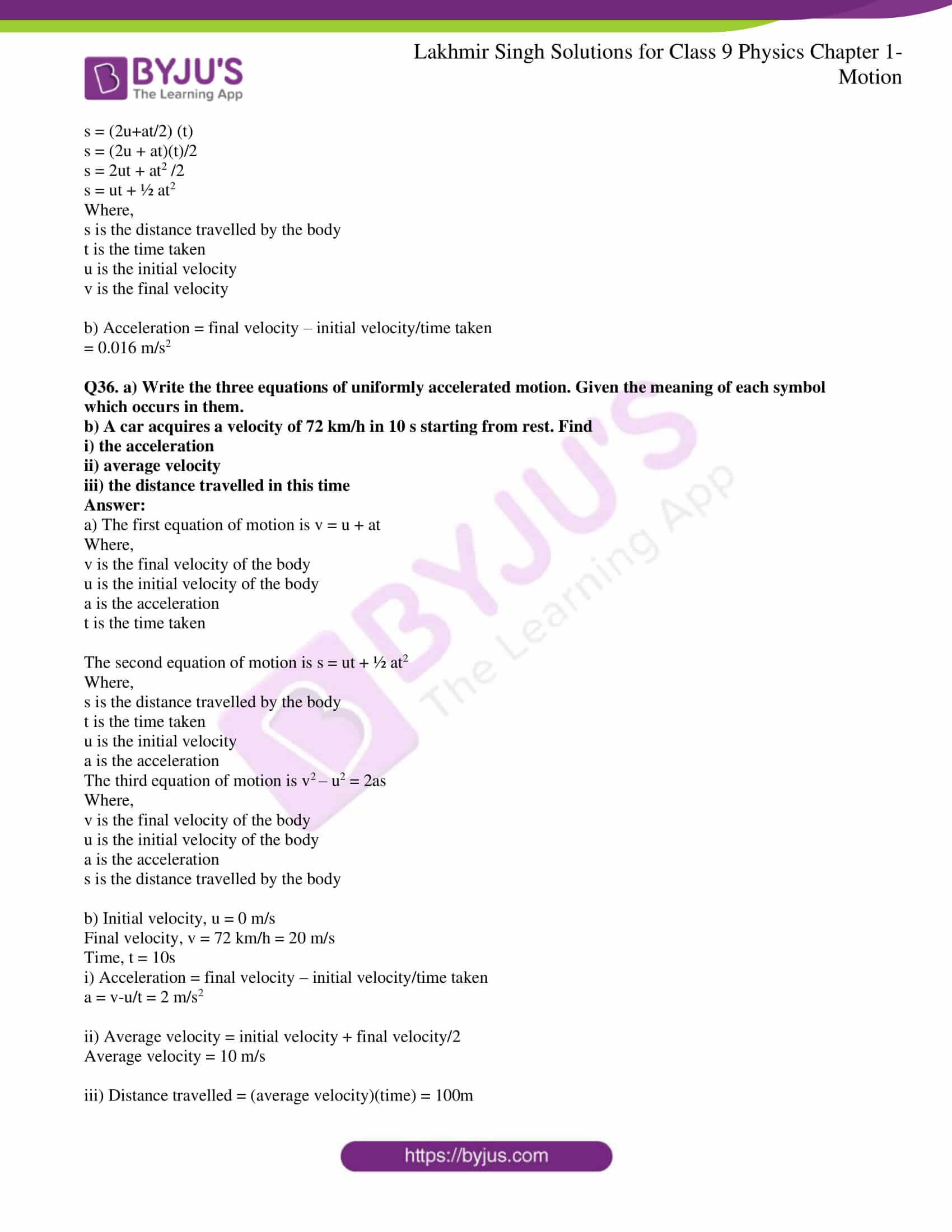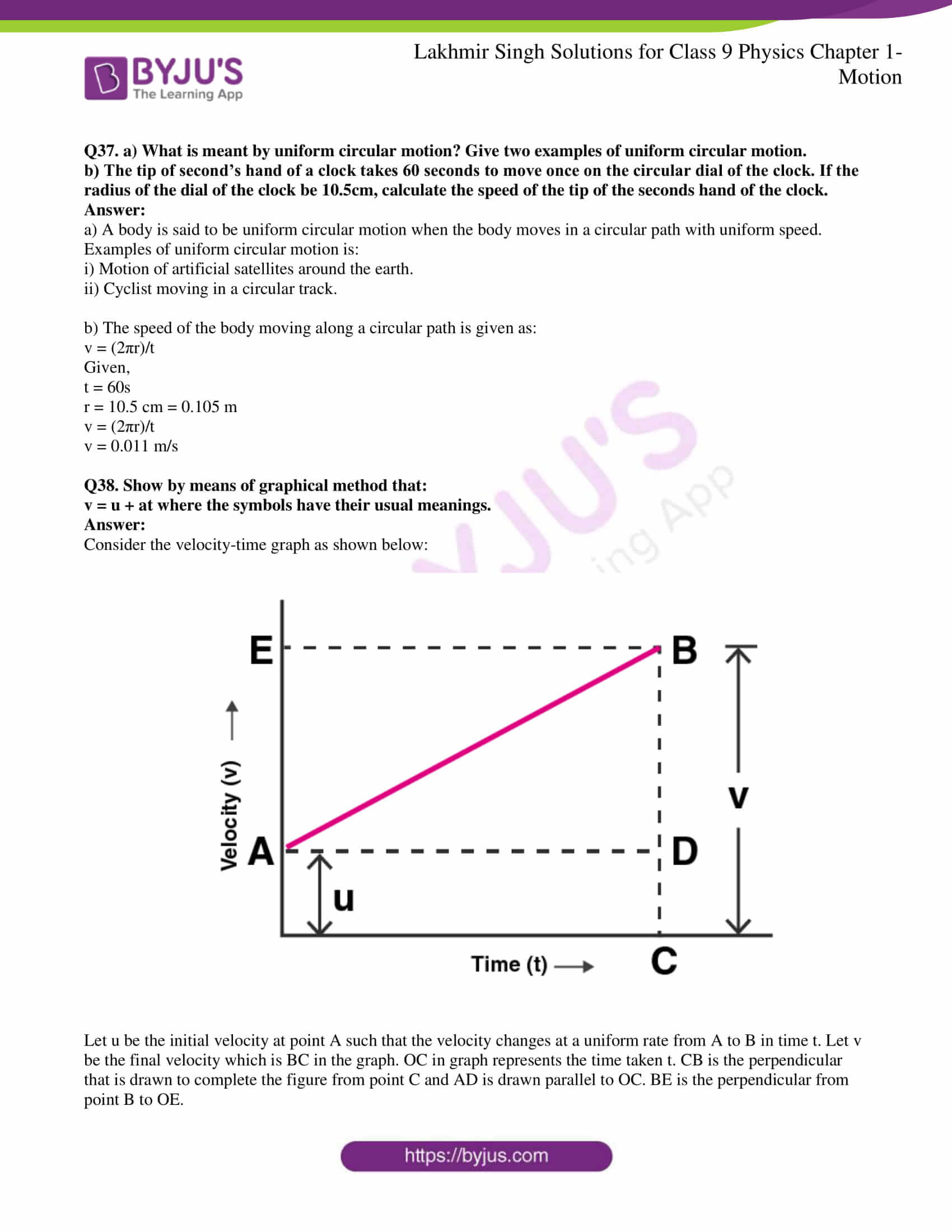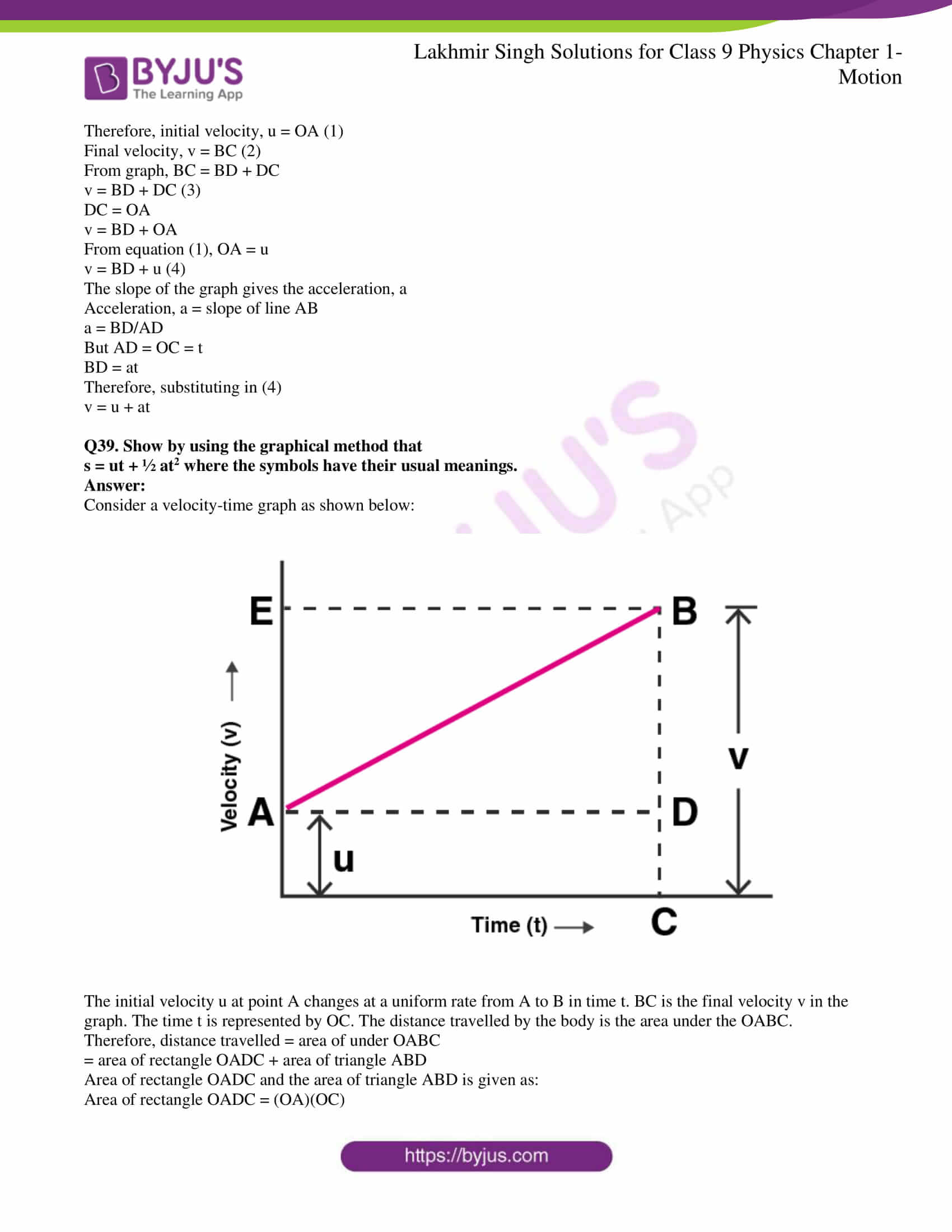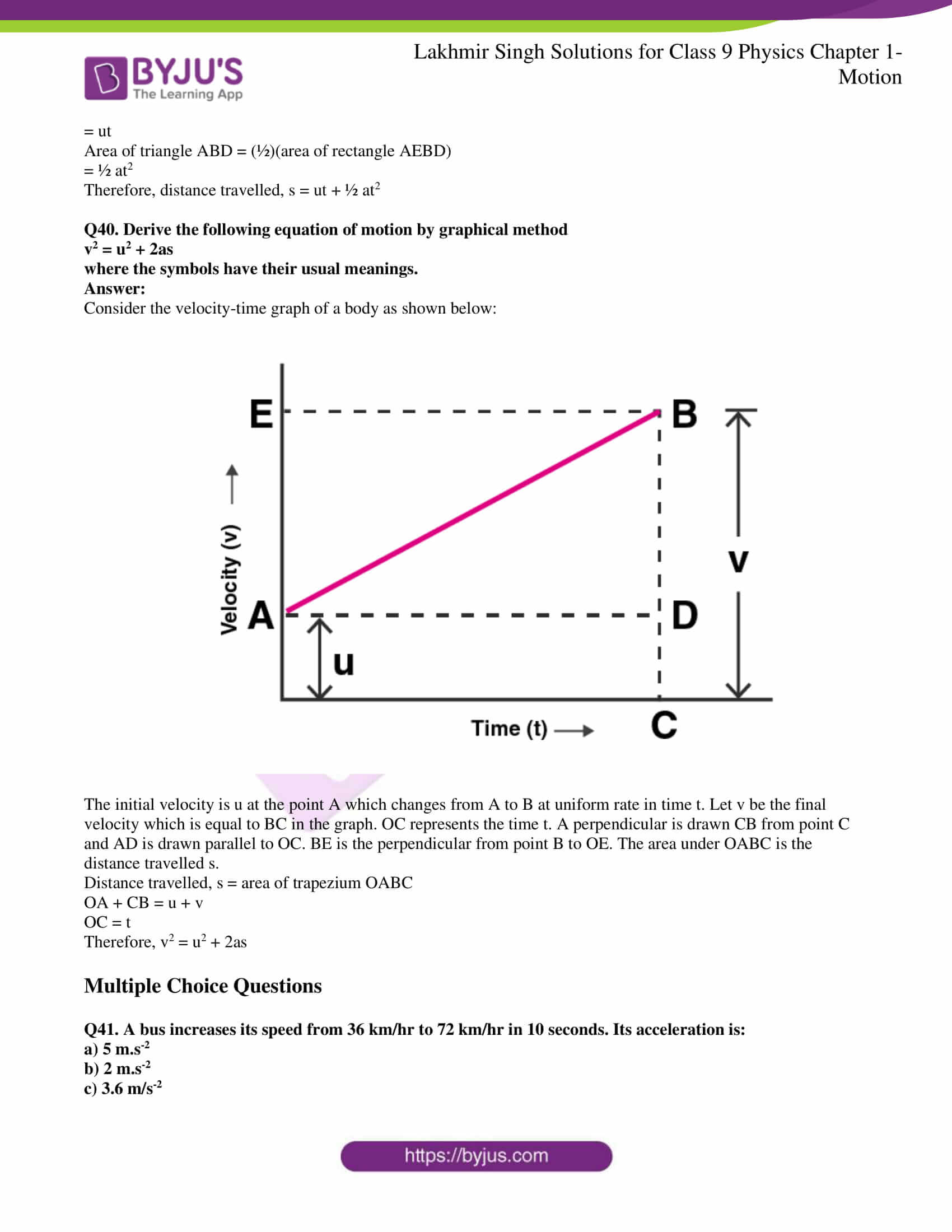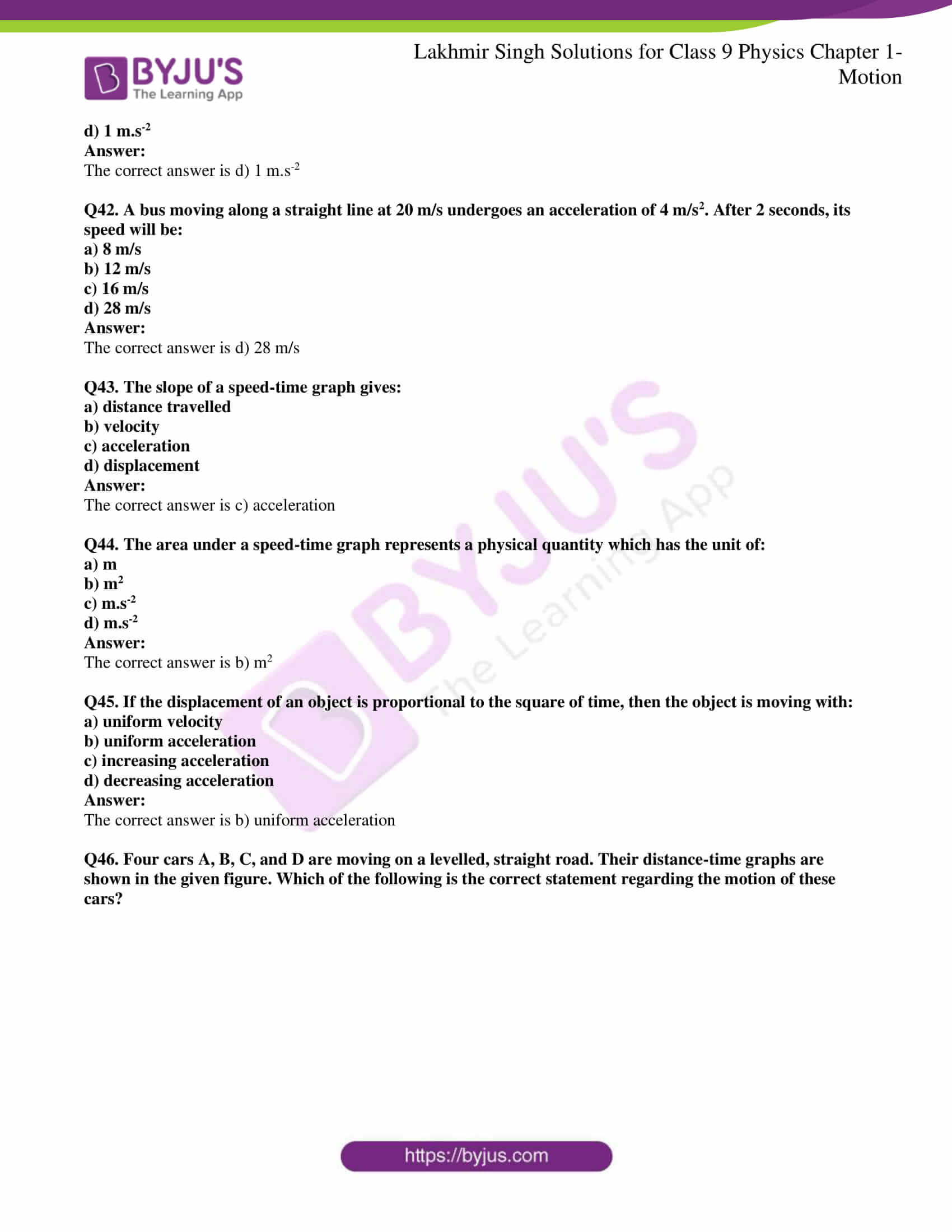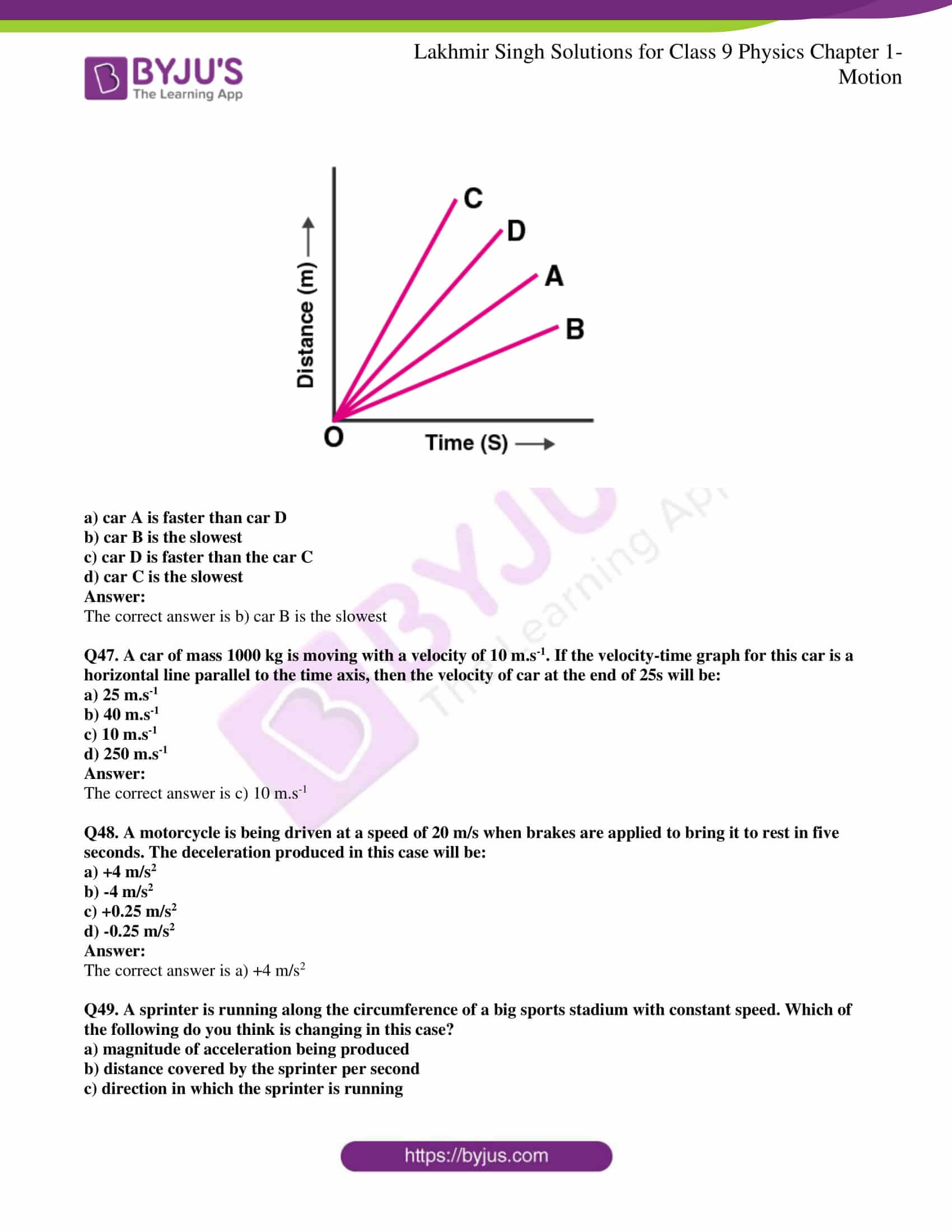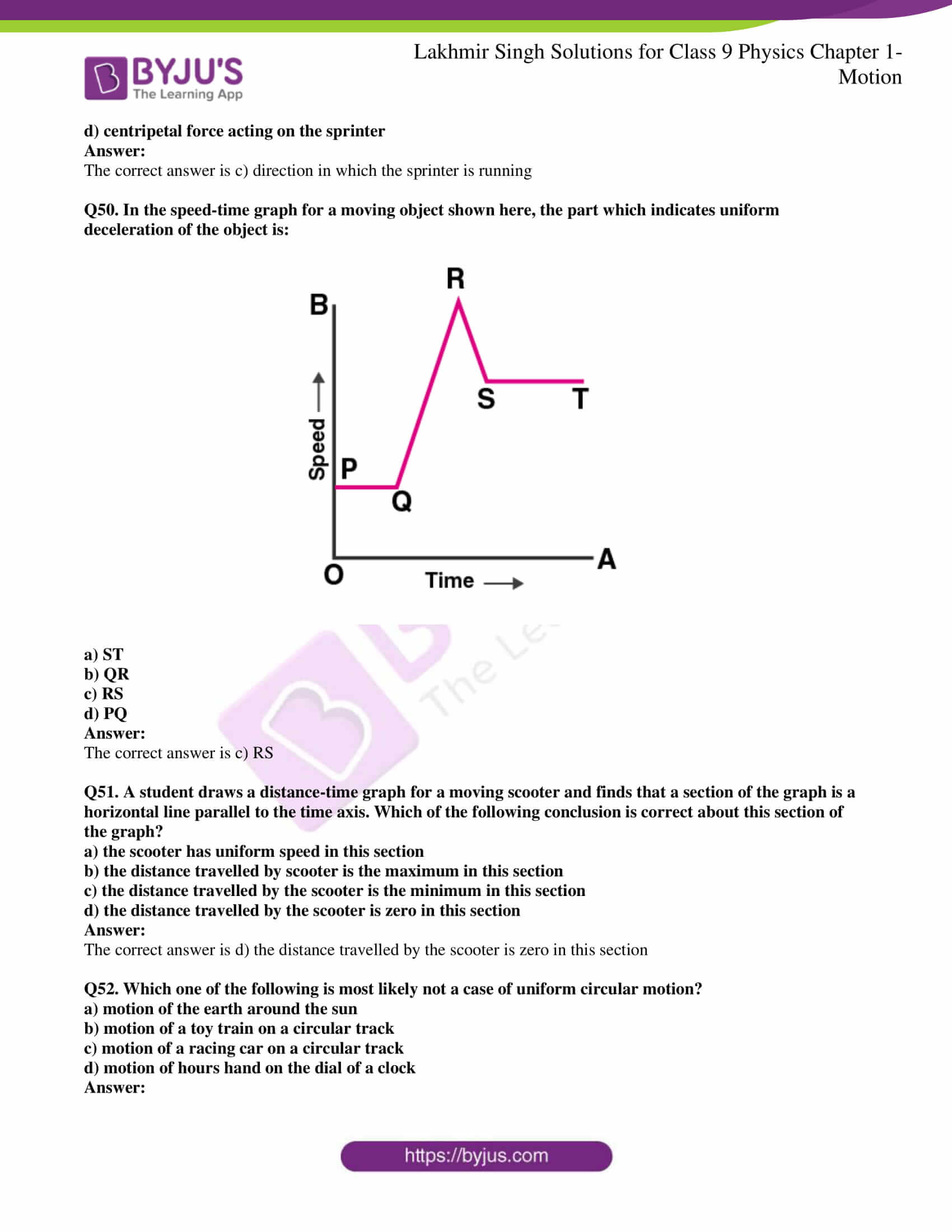### Access Lakhmir Singh Physics Class 9 Solutions For Chapter 1 Motion

Page No: 19

Q1. Is displacement a scalar quantity?

Displacement is not a scalar quantity, it is a vector quantity.

Q2. State whether distance is a scalar or a vector quantity.

Distance is a scalar quantity.

Q3. Change the speed of 6 m/s into km/h.

6 m/s = 6×(3600/1000) km/h = 21.6 km/h.

Q4. What name is given to the speed in a specified direction?

Velocity is the name that is given to the speed in a specified direction.

Q5. Give two examples of bodies having non-uniform motion.

a) Bus moving on the road

b) A racing horse motion

Q6. Name the physical quantity obtained by diving ‘distance travelled’ by ‘time taken’ to travel that distance.

Speed is the physical quantity that is obtained from distance travelled and time taken. Speed is defined as the distance travelled per unit time.

Q7. What do the following measure in a car?

a) speedometer

b) odometer

a) Speedometer in a car is used for measuring the instantaneous speed of the car.

b) Odometer in a car is used for recording the distance travelled by the car.

Q8. Name the physical quantity which gives us an idea of how slow or fast a body is moving.

To know how slow or fast the body is moving, speed is used.

Q9. Under what conditions can a body travel a certain distance and yet its resultant displacement be zero?

The resultant displacement of a body is said to be zero when the body comes back to its starting point and covers a certain non-zero distance.

Q10. In addition to speed, what else should we know to predict the position of a moving body?

Along with speed, the direction of the body should be known.

Q11. When is a body said to have uniform velocity?

A body is said to have a uniform velocity when it covers equal distances in equal intervals of time in a particular distance.

Q12. Under which condition is the magnitude of average velocity equal to average speed?

The magnitude of average velocity is equal to the average speed when the object moves in a single straight line.

Q13. Which of the two can be zero under certain conditions: average speed of a moving body or average velocity of a moving body?

The average velocity of a moving body can be zero.

Q14. Give one example of a situation in which a body has a certain average speed but its average velocity is zero.

When a car moves from parking area to the mall and back from mall to the parking area, the average speed is certain but the average velocity is zero.

Q15. What is the acceleration of a body moving with uniform velocity?

The acceleration of a body is zero that has a uniform velocity.

Q16. What is the other name of negative acceleration?

Negative acceleration is also known as retardation.

Q17. Name the physical quantity whose SI unit is:

a) m/s

b) m/s2

a) Speed

b) Acceleration

Q18. What type of motion is exhibited by a freely falling body?

Uniformly accelerated motion

Q19. What is the SI unit of retardation?

SI unit of retardation is m/s2.

Q20. Fill in the following blanks with suitable words:

a) Displacement is a ……… quantity whereas distance is a ……….

b) The physical quantity which gives both, the speed and direction of motion of a body is called its ……….

c) A motorcycle has a steady ………. of 3 m/s2. This means that every ……….. its …………. increases by ………….

d) Velocity is the rate of change of ……….. it is measured in ……….

e) Acceleration is the rate of change of ………. it is measured in …….

a) Vector and scalar

b) Velocity

c) Acceleration, second, velocity, 3m/s

d) Displacement, m/s

e) Velocity, m/s2

Q21. What type of motion, uniform or non-uniform is exhibited by a freely falling body? Give reason for your answer.

A freely falling body has a non-uniform motion because in the initial 1 second the distance covered is small and in the later 1 second the distance covered is large. As the distance covered is uneven, the motion is non-uniform.

Q22. State whether speed is a scalar or a vector quantity. Give reason for your choice.

Speed is a scalar quantity as it has only magnitude and there is no specific direction.

Q23. Bus X travels a distance of 360km in 5 hours whereas bus Y travels a distance of 476lm in 7 hours. Which bus is faster?

For bus X,

Speed = distance/time

Speed = 360/5 = 72 km/h

For bus Y,

Speed = 476/7 = 68 km/h

Therefore, the speed of bus X is more than the bus Y.

Q24. Arrange the following speeds in increasing order:

a) an athlete running with a speed of 10 m/s

b) a bicycle moving with a speed of 200 m/min

c) a scooter moving with a speed of 30 km/h

Speed of athlete = 10 m/s

Speed of bicycle = 200 m/min = 200/60 m/s = 3.33 m/s

Speed of scooter = 30 km/h = 30000/3600 m/s = 8.33 m/s

Therefore, bicycle < scooter < athlete

Q25. a) Write the formula for acceleration. Give the meaning of each symbol which occurs in it.

b) A train starting from railway station attains a speed of 21 m/s is one minute. Find its acceleration.

a) Acceleration = final velocity – initial velocity/ time taken

b) u = 0 m/s

v = 21 m/s

t = 1 min = 60s

a = v-u/t = 0.35 m/s2

Q26. a) What term is used to denote the change of velocity with time?

b) Give one word which means the same as ‘moving with a negative acceleration’.

c) The displacement of a moving object in a given interval of time is zero. Would the distance travelled by the object also be zero? Give reason for your answer.

a) Acceleration

b) Retardation

c) No, because when a body takes a round trip from its final position to the initial position, then the distance travelled will be a non-zero whereas the displacement is zero.

Q27. A snail covers a distance of 100 meters in 50 hours. Calculate the average speed of snail in km/h.

The snail covers a distance of 100 meters in 50 hours

The tortoise covers the same distance of 100 meters in 15 minutes

And this sprinter covers a distance of 100 meters in just 9.83 seconds.

Average speed = total distance travelled/total time taken

Total distance travelled = 100 m = 0.1 km

Total times taken = 50 h

Average speed = 0.1/50 = 0.002 km/h

Q28. A tortoise moves a distance of 100 meters in 15 minutes. What is the average speed of tortoise in km/h?

Total distance = 100m = 0.1 km

Total time taken = 15 minutes = 15/60 = 0.25 hour

Average speed = total distance travelled/total time taken = 0.4 km/h

Q29. If a sprinter runs a distance of 100 meters in 9.83 seconds, calculate his average speed in km/h.

Total distance travelled = 100m

Total time taken = 9.83 s

Average speed = total distance travelled/total time taken = 10.172 m/s

Average speed in km/h = 36.62 km/h

Q30. A motorcyclist drives from place A to B with a uniform speed of 30 km/h and returns from place B to A with a uniform speed of 20 km/h. Find his average speed.

Speed from A to B = 30 km/h

Let D be the distance from A to B

Time taken, T1 to travel from A to B = distance travelled/speed

T1 = D/30

Speed from B to A = 20 km/h

Time taken, T2 from B to a = distance travelled/speed

T2 = D/20

Total time taken, T = T1 + T2 = D/12

Total distance from A to B and B to A = 2D

Therefore, average speed = total distance travelled/total time taken = 24 km/h

Q31. A motorcyclist starts from rest and reaches a speed of 6 m/s after travelling with uniform acceleration for 3s. What is his acceleration?

Initial velocity = 0 m/s

Final velocity = 6 m/s

Time = 3 sec

a = (v-u)/t

a = 2 m/s2

Q32. An aircraft travelling at 600 km/h accelerates steadily at 10 km/h per second. Taking the speed of sound as 1100 km/h at the aircraft’s altitude how long will it take to reach the ‘sound barrier’?

Initial velocity, u = 600 km/h

Final velocity, v = 1100 km/h

Acceleration = 10 km/h/s = 600 km/h2

From formula,

a = (v-u)/t

t = (v-u)/a

t = 50 sec

Q33. If a bus travelling at 20 m/s is subjected to a steady deceleration of 5 m/s2, how long will it take to come to rest?

Deceleration, a = -5 m/s2

Initial velocity, u = 20 m/s

Final velocity, v = 0 m/s

Time, t = ?

a = (v-u)/t

-5 = (0-20)/t

t = 20/5 = 4s.

Q34. a) What is the difference between distance travelled by a body and its displacement? Explain with the help of a diagram.

b) An ant travels a distance of 8cm from P to Q and then moves a distance of 6cm at right angle to PQ. Find its resultant displacement.

a) Distance travelled is defined as the actual length covered by the body while displacement is the straight path between the initial and the final points. In the diagram below, a body moving from A to B and then B to C is the distance travelled while AB + BC is the displacement.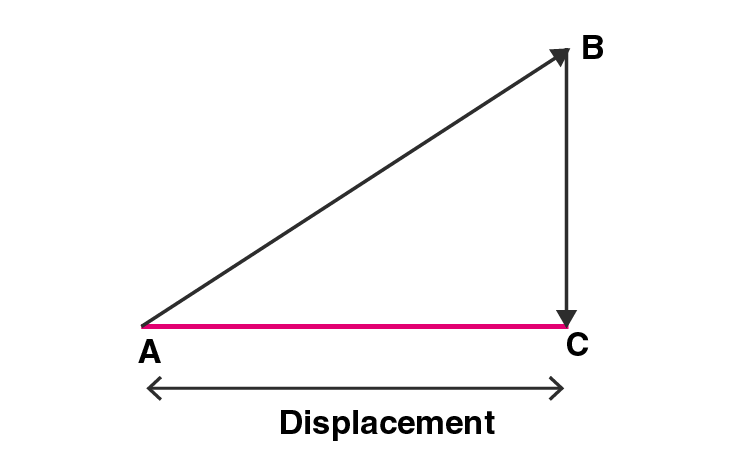b)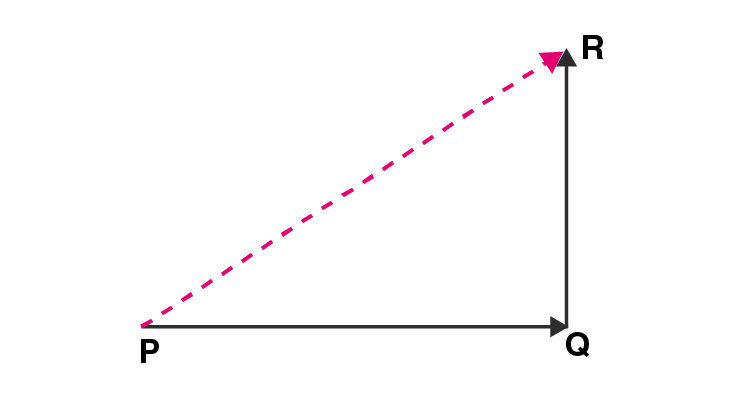PQ = 8cm

QR = 6cm

Resultant displacement is the square root of both = 10 cm

Q35. Define motion. What do you understand by the terms ‘uniform motion’ and ‘non-uniform motion’? Explain with examples.

Motion for a body is defined as the change in its position with respect to a body that is in rest which is taken as the point of reference.

A body is said to be in uniform motion when it covers an equal distances in equal intervals of time. Motion of free falling object is an example of uniform motion.

A body is said to be in non-uniform motion when it covers unequal distances in equal intervals of times. Athlete running a race.

Q36. a) Define speed. What is the SI unit of speed?

b) What is meant by:

i) average speed

ii) uniform speed?

a) Speed is defined as the distance travelled by the body in unit time. m/s is the SI unit of speed.

b) i) Average speed is the sum total of the distance travelled by a body divided by the total time taken to cover that distance.

Average speed = (total distance travelled)/(total time taken)

ii) Uniform speed refers to constant speed of a moving body in which the body covers an equal distance in equal time intervals.

Q37. a) Define velocity. What is the SI unit of velocity?

b) What is the difference between speed and velocity?

c) Convert a speed of 54 km/h into m/s.

a) Velocity is defined as the distance travelled by the body in unit time in a given direction. The SI unit of velocity is m/s.

b) The difference between speed and velocity is:

i) Speed is a scalar quantity while velocity is a vector quantity.

ii) Speed is always positive while velocity can be either positive or negative.

iii) Speed is defined as the distance travelled in unit time while velocity is the distance travelled in unit time in a particular direction.

c) Speed = 54 km/h = (54)(1000/3600) = 15 m/s

Q38. a) What is meant by the term acceleration? State the SI unit of acceleration.

b) Define the term ‘uniform acceleration’. Give one example of uniformly accelerated motion.

a) Acceleration is defined as the rate of change in a body’s velocity with respect to time. SI unit of acceleration is m/s2.

b) A body is said to have a uniform acceleration when it travels in a straight line with its velocity increasing at equal intervals of time. Motion of a free falling ball is an example of uniform acceleration.

Q39. The distance between Delhi and Agra is 200km. A train travels the first 100 km at a speed of 50 km/h. How fast must the train travel the next 100 km so as to average 70 km/h for the whole journey?

Total distance = 200 km

Average speed = 70 km/h

Total time taken = total distance/average speed = 200/70 = 20/7 h

First part of the journey,

Distance = 100 km

Speed = 50 km/h

Time taken, t1 = 100/50 = 2h

Second part of the journey,

Distance = 100 km

Speed = x km/h

Time taken, t2 = 100/x h

t1 + t2 = 20/7

x = 116.6 km/h

Q40. A train travels the first 15 km at a uniform speed of 30 km/h, the next 75 km at a uniform speed of 50 km/h and the last 10 km at a uniform speed of 20 km/h. Calculate the average speed for the entire train journey.

a) In the first part, speed of the train is 30 km/h for distance of 15 km.

Speed = distance/time

Time = distance/speed

T1 = ½ h

b) In the second part, speed of the train is 50 km/h for distance of 75 km.

T2 = 75/50 = 3/2 h

c) In the third part, speed of the train is 20 km/h for distance of 10 km.

T3 = 10/20 = ½ h

Therefore, the total distance covered = 100 km

Total time taken = 5/2 h

Average speed = total distance covered/total time taken = 40 km/h

Q41. A car is moving along a straight road at a steady speed. It travels 150 m in 5 seconds:

a) What is its average speed?

b) How far does it travel in 1 second?

c) How far does it travel in 6 seconds?

d) How long does it take to travel 240 m?

a) Average speed = total distance travelled/total time taken = 150/5 = 30 m/s

b) Time = 1 s

Distance = (average speed)(time) = 30 m/s

c) Time = 6 s

Distance = (average speed)(time) = 180m/s

d) Distance = 240m

Time = Distance/average speed = 240/30 = 8s

### Multiple Choice Questions

Q42. A particle is moving in a circular path of radius r. the displacement after half a circle would be:

a) 0

b) πr

c) 2r

d) 2πr

The correct answer is c) 2r

Q43. The numerical ratio of displacement to distance for a moving object is:

a) always less than 1

b) equal to 1 or more than 1

c) always more than 1

d) equal to 1 or less than 1

The correct answer d) equal to 1 or less than 1

Q44. A boy is sitting on a merry-go-round which is moving with a constant speed of 10 m.s-1. This means that the boy is:

a) at rest

b) moving with no acceleration

c) in accelerated motion

d) moving with uniform velocity

The correct answer c) in accelerated motion

Q45. In which of the following cases of motion, the distance moved and the magnitude of displacement are equal?

a) if the car is moving on straight road

b) if the car is moving on circular road

c) if the pendulum is moving to and fro

d) if a planet is moving around the sun

The correct answer a) if the car is moving on straight road

Q46. The speed of a moving object is determined to be 0.06 m/s. This speed is equal to:

a) 2.16 km/h

b) 1.08 km/h

c) 0.216 km/h

d) 0.0216 km/h

The correct answer c) 0.216 km/h

Q47. A freely falling object travels 4.9m in 1st second, 14.7m in 2nd second, 24.5m in 3rd second, and so on. This data shows that the motion of a freely falling object is a case of:

a) uniform motion

b) uniform acceleration

c) no acceleration

d) uniform velocity

The correct answer b) uniform acceleration

Q48. When a car runs on a circular track with a uniform speed, its velocity is said to be changing. This is because:

a) the car has a uniform acceleration

b) the direction of car varies continuously

c) the car travels unequal distances in equal time intervals

d) the car travels equal distances in unequal time intervals

The correct answer b) the direction of car varies continuously

Q49. Which of the following statement is correct regarding velocity and speed of a moving body?

a) velocity of a moving body is always higher than its speed

b) speed of a moving body is always higher than its velocity

c) speed of a moving body is its velocity in a given direction

d) velocity of a moving body is its speed in a given direction

The correct answer d) velocity of a moving body is its speed in a given direction

Q50. Which of the following can sometimes be ‘zero’ for a moving body?

i) average velocity ii) distance travelled iii) average speed iv) displacement

a) only i)

b) i) and ii)

c) i) and iv)

d) only iv)

The correct answer d) only iv)

Q51. When a car driver travelling at a speed of 10 m/s applies brakes and brings the car to rest in 20s, then retardation will be:

a) +2 m/s2

b) -2 m/s2

c) -0.5 m/s2

d) +0.5 m/s2

The correct answer d) +0.5 m/s2

Q52. Which of the following could not be a unit of speed?

a) km/h

b) s/m

c) m/s

d) mm.s-1

Q53. One of the following is not a vector quantity. This one is:

a) displacement

b) speed

c) acceleration

d) velocity

Q54. Which of the following could not be a unit of acceleration?

a) km/s2

b) cm.s-2

c) km/s

d) m/s2

Page No: 39

### Very Short Answer Type Questions

Q1. a) What remains constant in uniform circular motion?

b) What changes continuously in uniform circular motion?

a) Speed

b) Direction of motion

Q2. State whether the following statement is true or false:

Earth moves round the sun with uniform velocity.

False. The earth moves round the sun with uniform speed, but the velocity keeps changing.

Q3. A body goes round the sun with constant speed in a circular orbit. Is the motion uniform or accelerated?

The motion is accelerated.

Q4. What conclusion can you draw about the velocity of a body from the displacement-time graph shown below: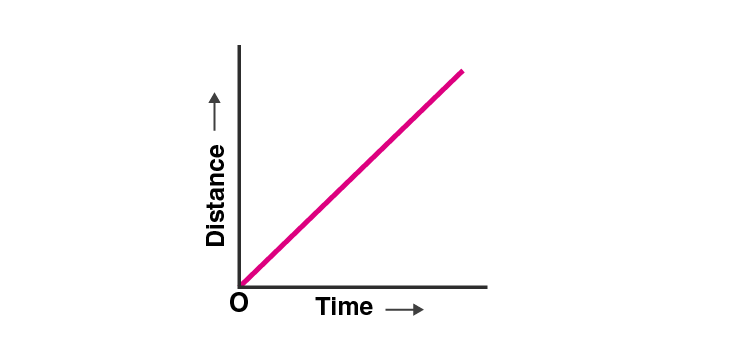The graph represents the uniform velocity.

Q5. Name the quantity which is measured by the area occupied under the velocity-time graph.

The area occupied under the velocity-time graph is the distance travelled by the moving body.

Q6. What does the slope of a speed-time graph indicate?

Acceleration is indicated by the slope of a speed-time graph.

Q7. What does the slope of a distance-time graph indicate?

Speed is indicated by the slope of a distance-time graph.

Q8. Give one example of a motion where an object does not change its speed but its direction of motion changes continuously.

Moon moving around the earth is an example.

Q9. Name the type of motion in which a body has a constant speed but not constant velocity.

Uniform circular motion

Q10. What can you say about the motion of a body if its speed-time graph is a straight line parallel to the time axis?

The speed of the body is constant or uniform.

Q11. What conclusion can you draw about the speed of a body from the following distance-time graph?The speed of a body is uniform.

Q12. What can you say about the motion of a body whose distance-time graph is a straight line parallel to the time axis?

When the distance-time graph is a straight line and parallel to the time axis, it is said to be stationary.

Q13. What conclusion can you draw about the acceleration of a body from the speed-time graph shown below?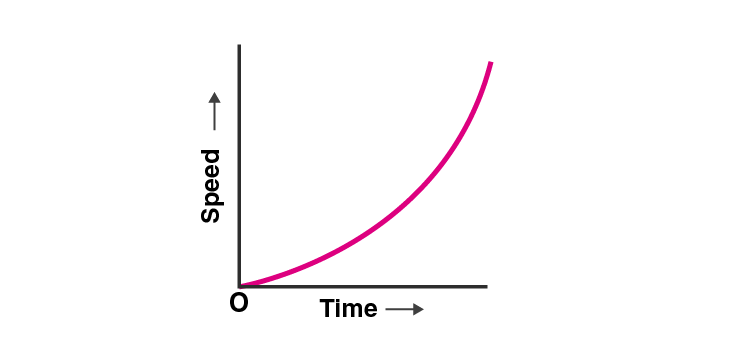The graph represents that the body is in non-uniform acceleration.

Q14. A satellite goes round the earth in a circular orbit with constant speed. Is the motion uniform or accelerated?This photograph shows a man-made ‘communications satellite’ going round the earth in a circular orbit. We can see the dish antennae, and solar panels clearly inn this photograph.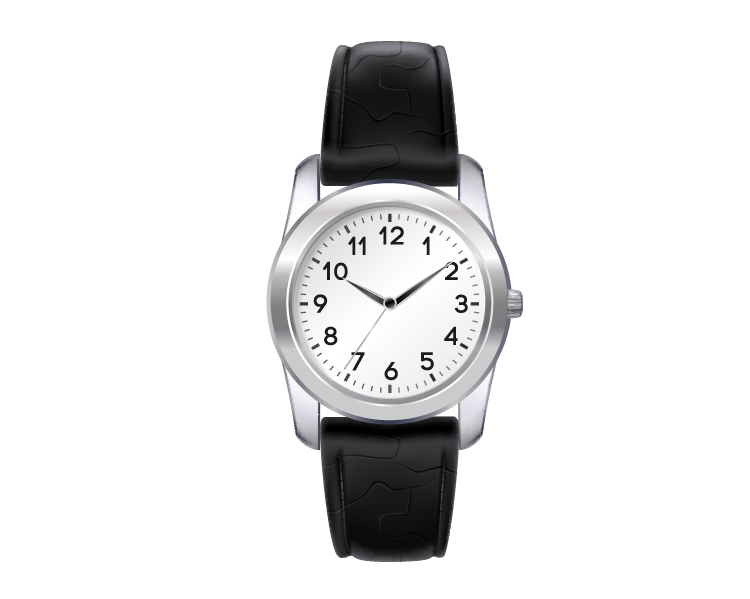This photograph shows a watch. The tip of seconds hand of this watch moves rapidly on the dial of the watch. The tips of minutes hand and hour hand also move on the dial but they move slowly.

In both the picture, the motion is accelerated as the velocity changes continuously.

Q15. What type of motion is represented by the tip of the seconds hand of a watch? Is it uniform or accelerated?

Uniform circular motion is represented by the tip of the seconds hand of a watch. It is an accelerated motion.

Q16. Fill in the following blanks with suitable words:

a) If a body moves with uniform velocity, its acceleration is ………..

b) The slope of a distance-time graph indicates ……………. of a moving body.

c) The slope of a speed-time graph of a moving body gives its ……….

d) In a speed-time graph, the area enclosed by the speed-time curve and the time axis gives the …….. by the body.

e) It is possible for something to accelerate bit not change its speed if it moves in a ………..

a) Zero

b) Speed

c) Acceleration

d) Distance travelled

e) Circular path

Q17. Is the uniform circular motion accelerated? Give reasons for your answer.

Yes, uniform circular motion is accelerated as the velocity changes due to continuous change in the direction of the motion.

Q18. Write the formula to calculate the speed of a body moving along a circular path. Give the meaning of each symbol which occurs in it.

The speed of a moving body along a circular path is given as:

v = (2πr)/t

Where,

v is the velocity

r is the radius of the circular path

t is the time taken to complete one round

Q19. Explain why the motion of a body which is moving with constant speed in a circular path is said to be accelerated.

The motion of a body which is moving with a constant speed in a circular path is said to be accelerated because the velocity of the body changes continuously along the direction of motion.

Q20. What is the difference between uniform linear motion and uniform circular motion? Explain with examples.

Uniform linear motion is the uniform motion along the linear path in which the direction of the motion is fixed. A car moving with uniform speed is an example of uniform linear motion.

Uniform circular motion is the uniform motion along the circular path in which the direction of the motion changes continuously. Motion of the earth around the sun is an example of uniform circular motion.

Q21. State an important characteristics of uniform circular motion. Name the force which brings about uniform circular motion.

An important characteristic of uniform circular motion is that the direction of motion changes continuously with time.

Centripetal force is responsible for the uniform circular motion.

Q22. Find the initial velocity of a car which is stopped in 10 seconds by applying brakes. The retardation due to brakes is 2.5 m/s2.

Initial velocity, u = ?

Final velocity, v = 0 m/s

Retardation, a = -2.5 m/s2

Time, t = 10s

v = u + at

u = 25 m/s

Q23. Describe the motion of a body which is accelerating at a constant rate of 10 m/s2. If the body starts from rest, how much distance will it cover in 2s?

Initial velocity, u = 0 m/s

Time, t = 2s

Acceleration, a = 10 m/s2

s = ut + ½ ats = 20m

Q24. A motorcycle moving with a speed of 5 m/s is subjected to an acceleration of 0.2 m/s2. Calculate the speed of the motorcycle after 10 seconds and the distance travelled in this time.

Initial velocity, u = 5 m/s

Final velocity, v = ?

Acceleration, a = 0.2 m/s2

Time, t = 10 s

v = u + at = 7 m/s

Q25. A bus running at a speed of 18 km/h is stopped in 2.5 seconds by applying brakes. Calculate the retardation produced.

Initial velocity, u = 18 km/h = 5 m/s

Final velocity, v = 0 m/s

Time, t = 2.5s

Retardation = 2 m/s2

Q26. A train starting from rest moves with a uniform acceleration of 0.2 m/s2 for 5 minutes. Calculate the speed acquired and the distance travelled in this time.

Initial velocity, u = 0 m/s

Final velocity, v = ?

Acceleration, a = 0.2 m/s2

Time, t = 5 min = 300 s

v = u + at = 60 m/s

Q27. Name the two quantities the slope of whose graph gives:

a) speed

b) acceleration

a) Distance and time

b) Speed and time

Q28. A cheetah starts from rest and accelerates at 2 m/s2 for 10 seconds. Calculate

a) the final velocity

b) the distance travelled

Initial velocity, u = 0 m/s

Final velocity, v = ?

Acceleration, a = 2 m/s2

Time, t = 10s

a) v = u + at = 20 m/s

b) s = ut + 1/2at2 = 100m

Q29. A train travelling at 20 m/s accelerates at 0.5 m/s2 for 30s. How far will it travel in this time?

Initial velocity, u = 20 m/s

Time, t = 30s

Acceleration, a = 0.5 m/s2

s = ut = ½ at2 = 825m

Q30. A cyclist is travelling at 15 m/s. She applies brakes so that she does not collide with a wall 18 m away. What deceleration must she have?

Initial velocity, u = 15 m/s

Final velocity, v = 0 m/s

Distance, s = 18m

Acceleration, a = ?

Deceleration, v2 – u2 = 2as = 6.25 m/s2

Q31. Draw a velocity-time graph to show the following motion:

A car accelerates uniformly from rest for 5s then it travels at a steady velocity 5s.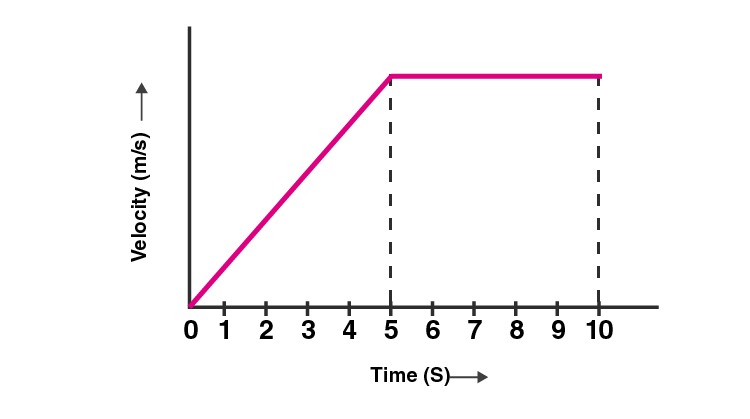Q32. The velocity-time graph for part of a train journey is a horizontal straight line. What does this tell you about

a) the train’s velocity

a) The train has a uniform velocity

b) There is no acceleration

Q33. a) Explain the meaning of the following equation of motion: v = u + at where the symbols have their usual meanings.

b) A body starting from rest travels with uniform acceleration. If it travels 100 m in 5 s what is the value of acceleration?

a) v = u + at is the first equation of motion.

Where,

v is the final velocity

u is the initial velocity

a is the acceleration

t is the time taken

b) Initial velocity, u = 0 m/s

Time, t = 5s

Distance, s = 100 m

Acceleration, a = ?

s = ut + 1/2at2

a = 200/25 = 8 m/s2

Q34. a) Derive the formula: v = u + at where the symbols have usual meanings

b) A bus was moving with a speed of 54 km/h. On applying brakes it stopped in 8 seconds. Calculate the acceleration.

a) Let u be the initial velocity of the body and v be the final velocity of the body. The body is accelerated uniformly with which is represented by a. The time taken is t. Therefore, the equation v = u + at.

Acceleration = change in velocity/time taken

a = (v-u)/t

at = v – u

v = u + at

b) Initial velocity, u = 54 km/h = 15 m/s

Final velocity, v = 0 m/s

Time, t = 8s

Acceleration, a = ?

a = (v-u)/t = -1.87 m/s2

Q35. a) Derive the formula: s = ut + ½ at 2.

b) A train starting from stationary position and moving with uniform acceleration attains a speed of 36km per hour in 10 minutes. Find its acceleration.

a) Average velocity = (initial velocity + final velocity)/2

= u + v/2

Distance travelled = (average velocity ) (time)

s = (u+v/2) (t)

s = (2u+at/2) (t)

s = (2u + at)(t)/2

s = 2ut + at2 /2

s = ut + ½ at2

Where,

s is the distance travelled by the body

t is the time taken

u is the initial velocity

v is the final velocity

b) Acceleration = final velocity – initial velocity/time taken

= 0.016 m/s2

Q36. a) Write the three equations of uniformly accelerated motion. Given the meaning of each symbol which occurs in them.

b) A car acquires a velocity of 72 km/h in 10 s starting from rest. Find

i) the acceleration

ii) average velocity

iii) the distance travelled in this time

a) The first equation of motion is v = u + at

Where,

v is the final velocity of the body

u is the initial velocity of the body

a is the acceleration

t is the time taken

The second equation of motion is s = ut + ½ at2

Where,

s is the distance travelled by the body

t is the time taken

u is the initial velocity

a is the acceleration

The third equation of motion is v2 – u2 = 2as

Where,

v is the final velocity of the body

u is the initial velocity of the body

a is the acceleration

s is the distance travelled by the body

b) Initial velocity, u = 0 m/s

Final velocity, v = 72 km/h = 20 m/s

Time, t = 10s

i) Acceleration = final velocity – initial velocity/time taken

a = v-u/t = 2 m/s2

ii) Average velocity = initial velocity + final velocity/2

Average velocity = 10 m/s

iii) Distance travelled = (average velocity)(time) = 100m

Q37. a) What is meant by uniform circular motion? Give two examples of uniform circular motion.

b) The tip of second’s hand of a clock takes 60 seconds to move once on the circular dial of the clock. If the radius of the dial of the clock be 10.5cm, calculate the speed of the tip of the seconds hand of the clock.

a) A body is said to be uniform circular motion when the body moves in a circular path with uniform speed. Examples of uniform circular motion is:

i) Motion of artificial satellites around the earth.

ii) Cyclist moving in a circular track.

b) The speed of the body moving along a circular path is given as:

v = (2πr)/t

Given,

t = 60s

r = 10.5 cm = 0.105 m

v = (2πr)/t

v = 0.011 m/s

Q38. Show by means of graphical method that:

v = u + at where the symbols have their usual meanings.

Consider the velocity-time graph as shown below: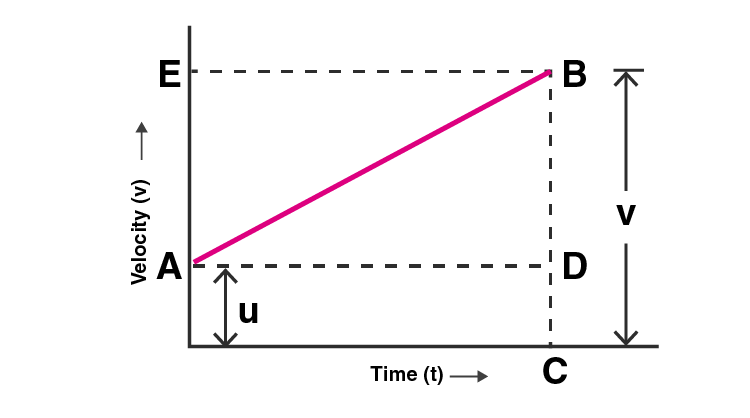Let u be the initial velocity at point A such that the velocity changes at a uniform rate from A to B in time t. Let v be the final velocity which is BC in the graph. OC in graph represents the time taken t. CB is the perpendicular that is drawn to complete the figure from point C and AD is drawn parallel to OC. BE is the perpendicular from point B to OE.

Therefore, initial velocity, u = OA (1)

Final velocity, v = BC (2)

From graph, BC = BD + DC

v = BD + DC (3)

DC = OA

v = BD + OA

From equation (1), OA = u

v = BD + u (4)

The slope of the graph gives the acceleration, a

Acceleration, a = slope of line AB

But AD = OC = t

BD = at

Therefore, substituting in (4)

v = u + at

Q39. Show by using the graphical method that

s = ut + ½ at2 where the symbols have their usual meanings.

Consider a velocity-time graph as shown below:The initial velocity u at point A changes at a uniform rate from A to B in time t. BC is the final velocity v in the graph. The time t is represented by OC. The distance travelled by the body is the area under the OABC.

Therefore, distance travelled = area of under OABC

= area of rectangle OADC + area of triangle ABD

Area of rectangle OADC and the area of triangle ABD is given as:

Area of rectangle OADC = (OA)(OC)

= ut

Area of triangle ABD = (½)(area of rectangle AEBD)

= ½ at2

Therefore, distance travelled, s = ut + ½ at2

Q40. Derive the following equation of motion by graphical method

v2 = u2 + 2as

where the symbols have their usual meanings.

Consider the velocity-time graph of a body as shown below:The initial velocity is u at the point A which changes from A to B at uniform rate in time t. Let v be the final velocity which is equal to BC in the graph. OC represents the time t. A perpendicular is drawn CB from point C and AD is drawn parallel to OC. BE is the perpendicular from point B to OE. The area under OABC is the distance travelled s.

Distance travelled, s = area of trapezium OABC

OA + CB = u + v

OC = t

Therefore, v2 = u2 + 2as

### Multiple Choice Questions

Q41. A bus increases its speed from 36 km/hr to 72 km/hr in 10 seconds. Its acceleration is:

a) 5 m.s-2

b) 2 m.s-2

c) 3.6 m/s-2

d) 1 m.s-2

The correct answer is d) 1 m.s-2

Q42. A bus moving along a straight line at 20 m/s undergoes an acceleration of 4 m/s2. After 2 seconds, its speed will be:

a) 8 m/s

b) 12 m/s

c) 16 m/s

d) 28 m/s

The correct answer is d) 28 m/s

Q43. The slope of a speed-time graph gives:

a) distance travelled

b) velocity

c) acceleration

d) displacement

The correct answer is c) acceleration

Q44. The area under a speed-time graph represents a physical quantity which has the unit of:

a) m

b) m2

c) m.s-2

d) m.s-2

The correct answer is b) m2

Q45. If the displacement of an object is proportional to the square of time, then the object is moving with:

a) uniform velocity

b) uniform acceleration

c) increasing acceleration

d) decreasing acceleration

The correct answer is b) uniform acceleration

Q46. Four cars A, B, C, and D are moving on a levelled, straight road. Their distance-time graphs are shown in the given figure. Which of the following is the correct statement regarding the motion of these cars?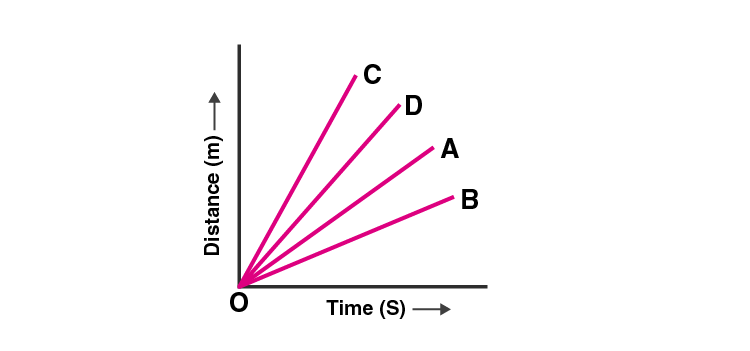a) car A is faster than car D

b) car B is the slowest

c) car D is faster than the car C

d) car C is the slowest

The correct answer is b) car B is the slowest

Q47. A car of mass 1000 kg is moving with a velocity of 10 m.s-1. If the velocity-time graph for this car is a horizontal line parallel to the time axis, then the velocity of car at the end of 25s will be:

a) 25 m.s-1

b) 40 m.s-1

c) 10 m.s-1

d) 250 m.s-1

The correct answer is c) 10 m.s-1

Q48. A motorcycle is being driven at a speed of 20 m/s when brakes are applied to bring it to rest in five seconds. The deceleration produced in this case will be:

a) +4 m/s2

b) -4 m/s2

c) +0.25 m/s2

d) -0.25 m/s2

The correct answer is a) +4 m/s2

Q49. A sprinter is running along the circumference of a big sports stadium with constant speed. Which of the following do you think is changing in this case?

a) magnitude of acceleration being produced

b) distance covered by the sprinter per second

c) direction in which the sprinter is running

d) centripetal force acting on the sprinter

The correct answer is c) direction in which the sprinter is running

Q50. In the speed-time graph for a moving object shown here, the part which indicates uniform deceleration of the object is:a) ST

b) QR

c) RS

d) PQ

The correct answer is c) RS

Q51. A student draws a distance-time graph for a moving scooter and finds that a section of the graph is a horizontal line parallel to the time axis. Which of the following conclusion is correct about this section of the graph?

a) the scooter has uniform speed in this section

b) the distance travelled by scooter is the maximum in this section

c) the distance travelled by the scooter is the minimum in this section

d) the distance travelled by the scooter is zero in this section

The correct answer is d) the distance travelled by the scooter is zero in this section

Q52. Which one of the following is most likely not a case of uniform circular motion?

a) motion of the earth around the sun

b) motion of a toy train on a circular track

c) motion of a racing car on a circular track

d) motion of hours hand on the dial of a clock

The correct answer is c) motion of a racing car on a circular track

Also, visit Lakhmir Singh Solutions for Class 9 Physics to get complete solutions for all chapters.

1. Keep it up!

2. Dhananjay Dubey

Achha hai

3. Mansi Dayanand Lokhande

Nice

4. Deepika

Thank uuu for these solutions 🥳